## Efficient Programming

Efficiency comes in two flavors:

1. Programming cost
2. Execution cost (time and memory)

### Terms

Module: A set of methods that work together as a whole to perform some task or set of related tasks.

Encapsulated: A module is said to be encapsulated if its implementation is completely hidden, and it can be accessed only through a documented interface.

API: An API (Application Programming Interface) of an ADT is the list of constructors and methods and a short description of each.

ADT: ADT’s (Abstract Data Type) are high-level types that are defined by their behaviors, not their implementations.

Deque in Proj1 was an ADT that had certain behaviors (addFirst, addLast, etc.). But, the data structures we actually used to implement it were ArrayDeque and LinkedListDeque

Some ADT’s are actually special cases of other ADT’s. For example, Stacks and Queues are just lists that have even more specific behavior.

### 3 Ways To Implement

1. Extension (Inheriting)

Borrow the methods from LinkedList and use them as its own.

2. Delegation (Passing in a class)

Create a Linked List object and call its methods.

3. Adapter

Similar to delegation, but it can use any class that implements the List interface (Linked List, ArrayList, etc.)

Delegation vs. Extension: Right now it may seem that Delegation and Extension are pretty much interchangeable; however, there are some important differences that must be remembered when using them.

Extension tends to be used when you know what is going on in the parent class. In other words, you know how the methods are implemented. Additionally, with extension, you are basically saying that the class you are extending from acts similarly to the one that is doing the extending.

On the other hand, Delegation is when you do not want to consider your current class to be a version of the class that you are pulling the method from.

### Idea of “View”

Views: Views are an alternative representation of an existed object. Views essentially limit the access that the user has to the underlying object. However, changes done through the views will affect the actual object.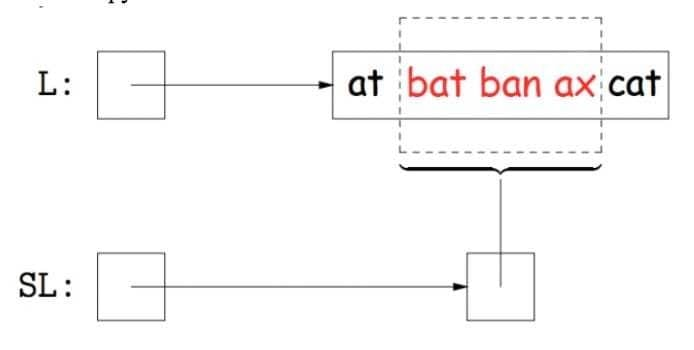Issue: How do you return an actual List but still have it affect another List? Access methods

Usage:

An observation that should be made is that getting the k-th item from our subList is the same as getting the k-th item from our original list with an offset equal to our start index. Because we are using a get method of our outer class (the most parent one) we change our original list.

Similarly, adding an element to our subList is the same as adding an element to our original list with an offset equal to the start index of the subList.

### The Takeaway

APIs are pretty hard to design; however, having a coherent design philosophy can make your code much cleaner and easier to deal with.

Inheritance is tempting to use frequently, but it has problems and should be used sparingly, only when you are certain about attributes of your classes (both those being extended and doing the extending).

## Asymptotics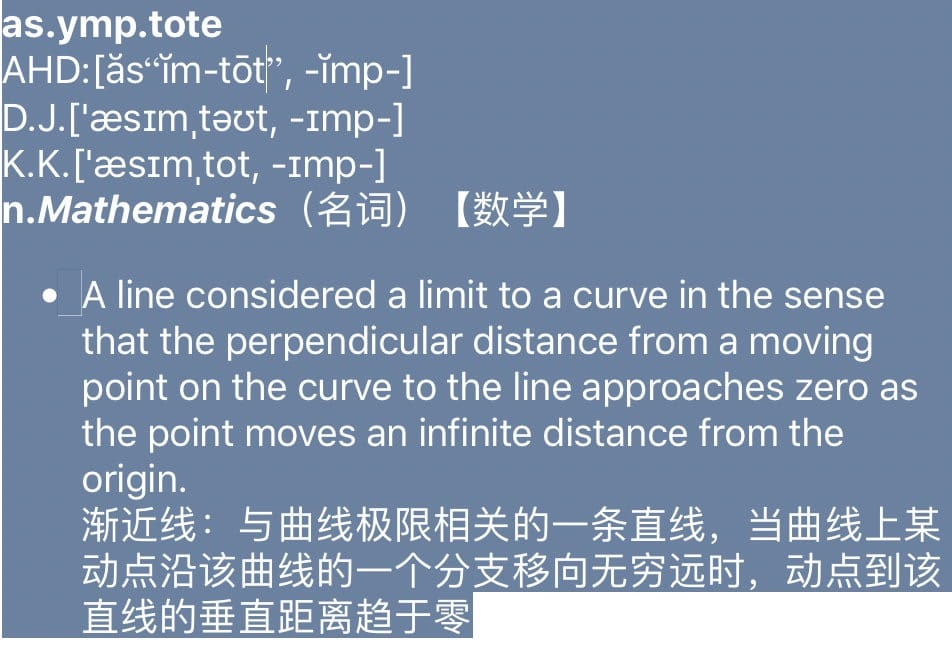Technique 2B: Count possible operations in terms of input array size N.

• Good: Machine independent. Input dependence captured in model. Tells you how algorithm scales.
• Bad: Even more tedious to compute. Doesn’t tell you actual time.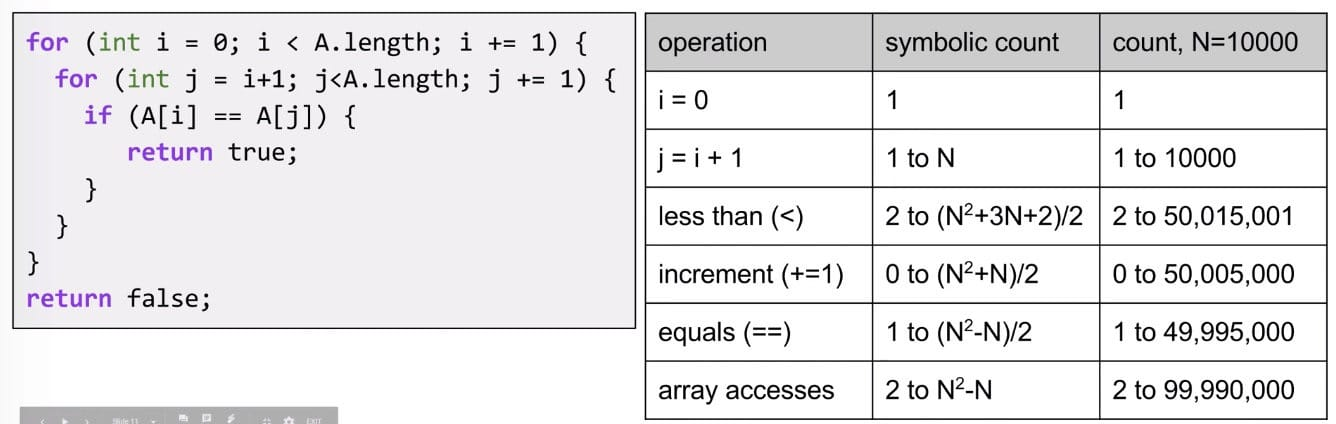What about the operation of +1 or -1?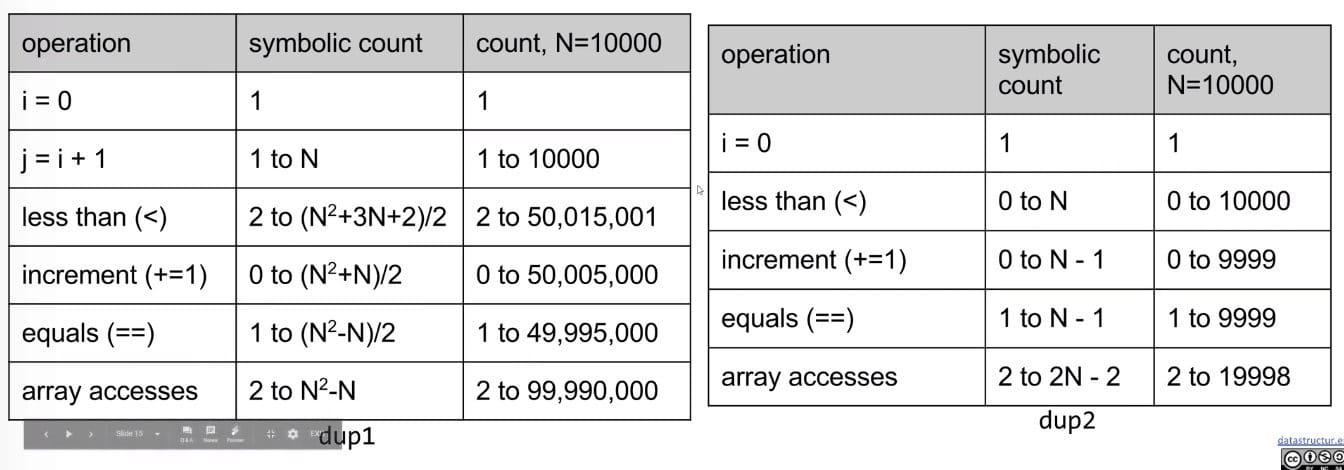Which one is better?

• An answer: It takes fewer operations to accomplish the same goal.
• Better answer: Algorithm scales better in the worst case $\frac{(N^2 + 3N + 2)}{2}$ vs. $N$
• Even better answer: Parabolas $N^2$ grow faster than lines $N$.
• Note: This is the same idea as our “better” answer, but it provides a more general geometric intuition.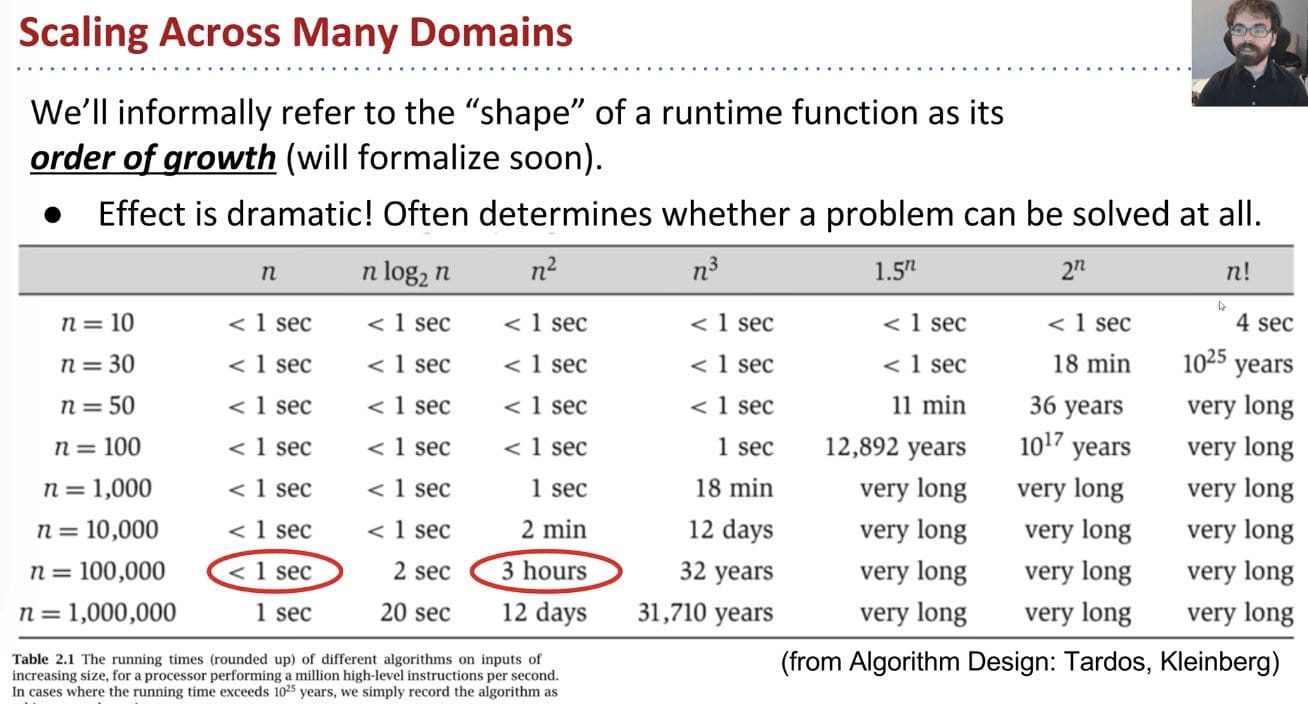### Simplification

Convert the table into worst case o.o.g. (order of growth):

• Simplification 1: Consider only the Worst Case

When comparing algorithms, we often only care about the worst case (though we’ll see some exceptions later in this course).

• $N$ [linear]
• $N^2$ [quadratic]
• $N^3$ [cubic]
• $N^6$ [sextic]

$\alpha (100 N^2 + 3N)+\beta (2 N^3 + 1) + 5000\gamma$

For very large N, the $2\beta N^3$ term is much larger than the others.

• Simplification 2: Restrict Attention to One Operation

Pick some representative operation to act as a proxy for overall runtime. The operation we choose can be called the cost model.

• Good choice: increment, or < or ==, or array accesses.
• Bad choice: assignment of j = i + 1, or i = 0 (since they execute only once).
• Simplification 3: Eliminate Low Order Terms

Tilde Notation (~)

Ignore lower order terms!

• Simplification 4: Eliminate Multiplicative Constants

Ignore multiplicative constants.

Why? No real meaning! Remember that by choosing a single representative operation, we already “threw away” some information.

Summary:

1. Only consider the worst case
2. Pick a representative operation (aka: cost model)
3. Ignore lower order terms
4. Ignore multiplicative constants

However, rather than building the entire table (it means counting), we can instead:

1. Choose our cost model (representative operation we want to count).
2. Figure out the order of growth for the count of our representative operation by either:
• Making an exact count, and discarding unnecessary pieces
• Or, using intuition / inspection to determine orders of growth (comes with practice!)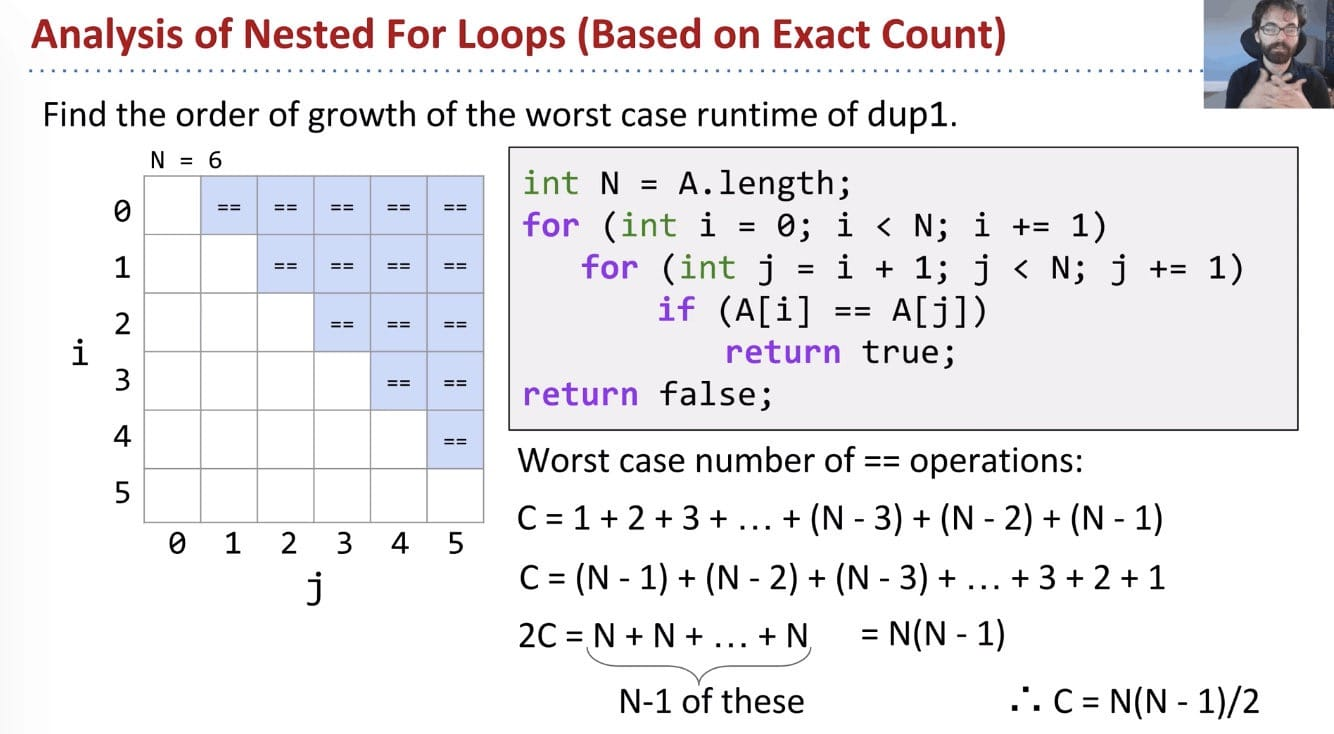### Big Theta - $\Theta(N)$

Given a piece of code, we can express its runtime as a function $R(N)$. Rather than finding $R(N)$ exactly, we instead usually only care about the order of growth of $R(N)$.

Big Theta $\Theta$ is exactly equivalent to order of growth.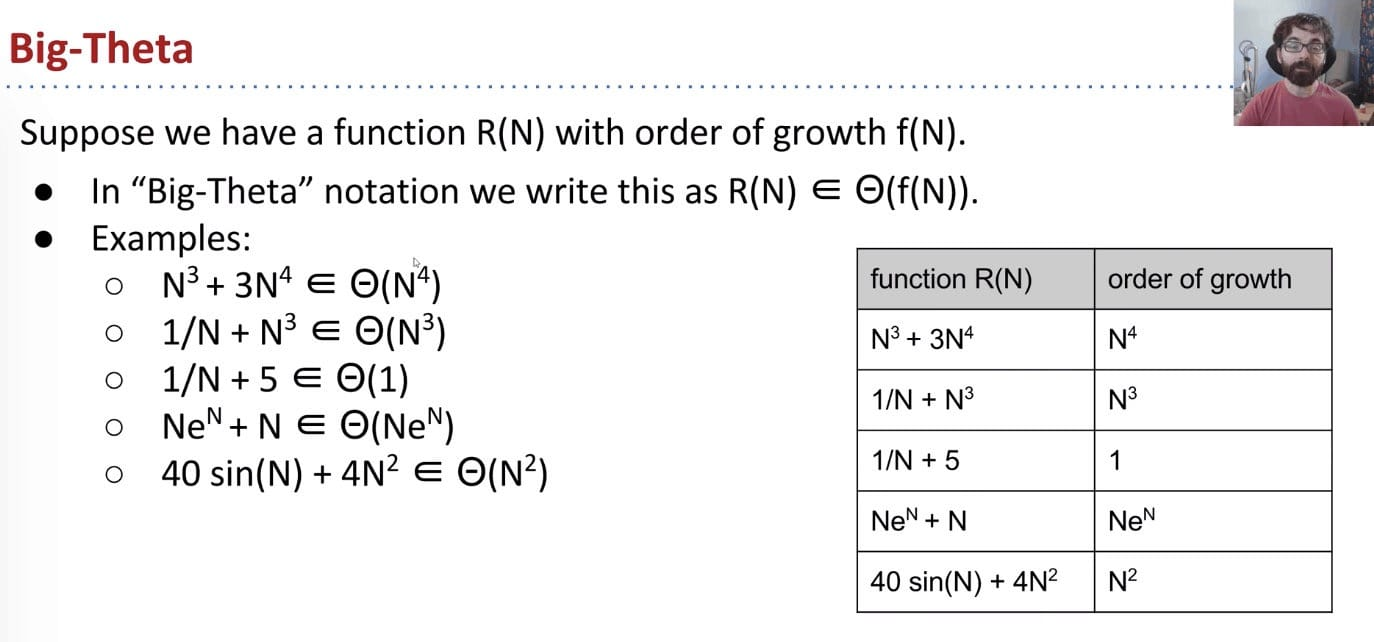Big-Theta Formal Definition: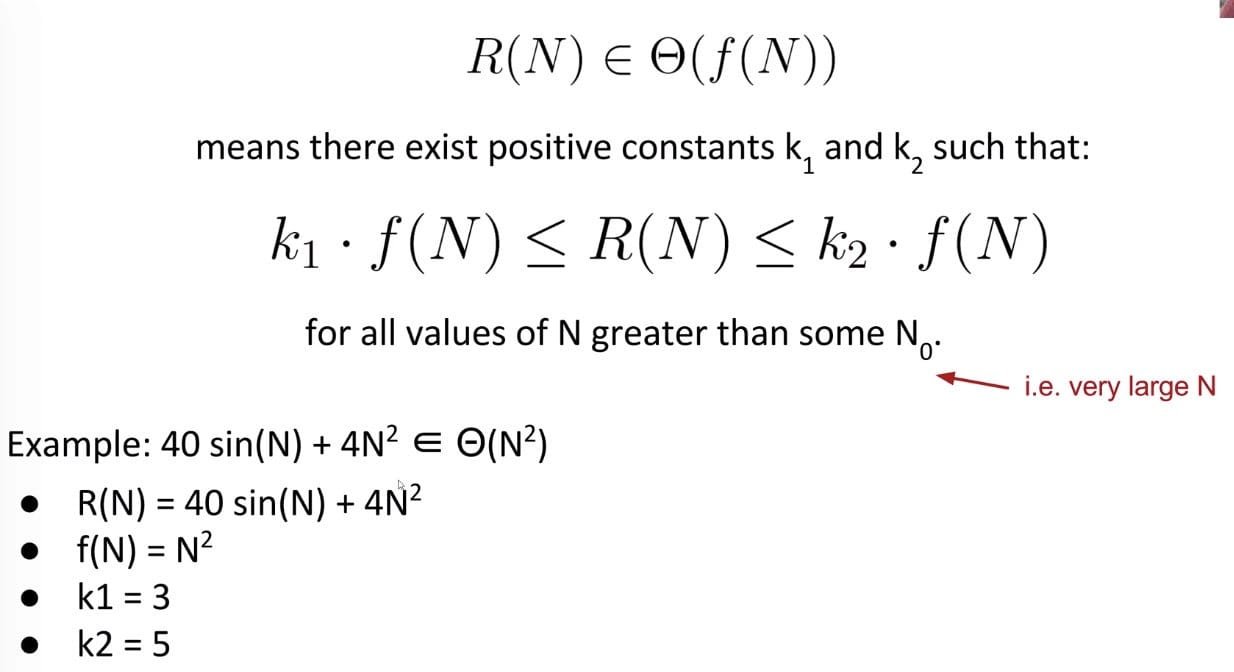$R(N)$ is both upper and lower bounded by $\Theta(f(N))$.

Using Big-Theta doesn’t change anything about runtime analysis (no need to find k1 or k2 or anything like that)

The only difference is that we use $\Theta$ symbol anywhere we would have said “order of growth”.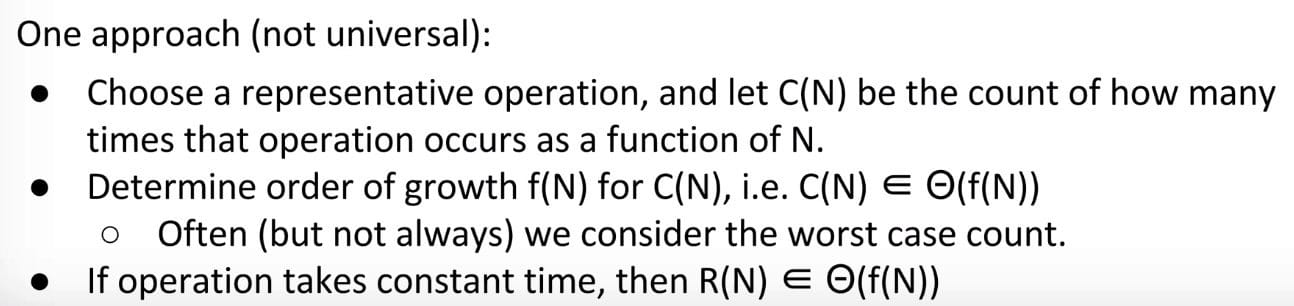### Big O - $O(N)$

We use Big Theta to describe the order of growth of a function, or the rate of growth of the runtime of a piece of code.

For example, binary search on $N$ items has worst case runtime of $\Theta(log N)$

Whereas Big Theta can informally be thought of as something like equals, Big O can be thought of as less than or equal.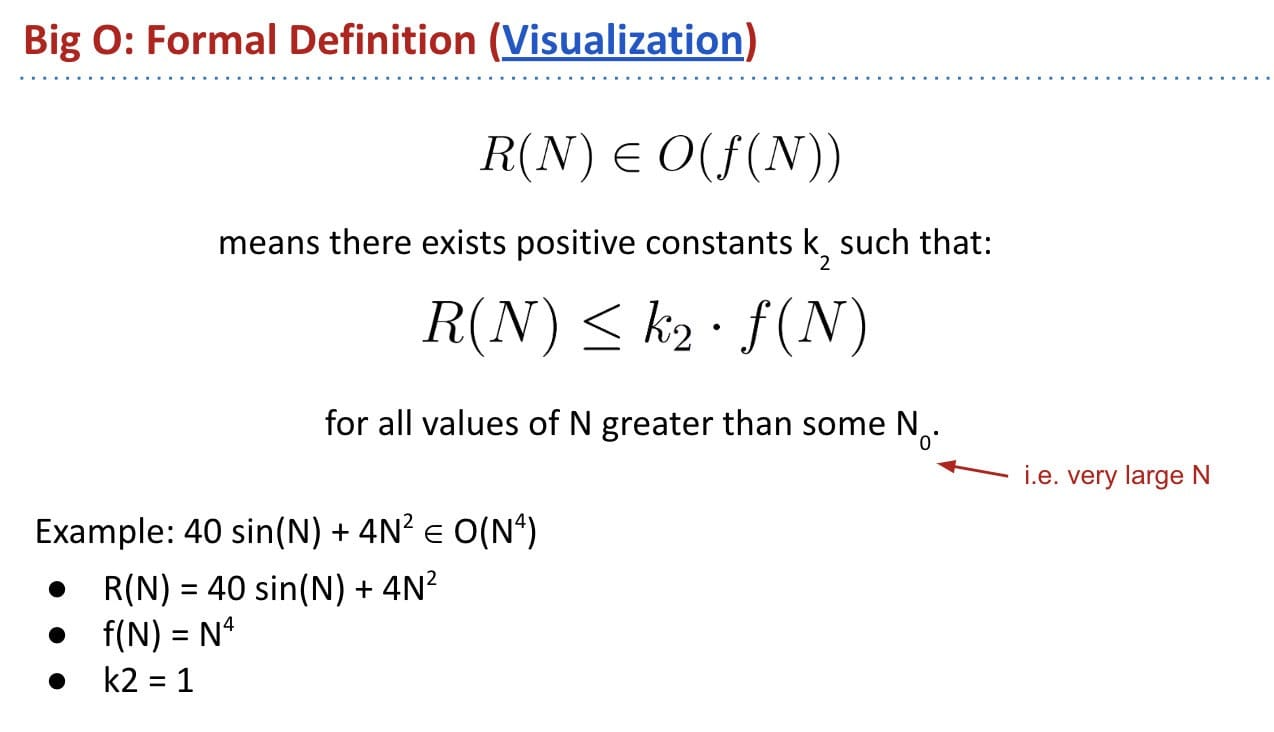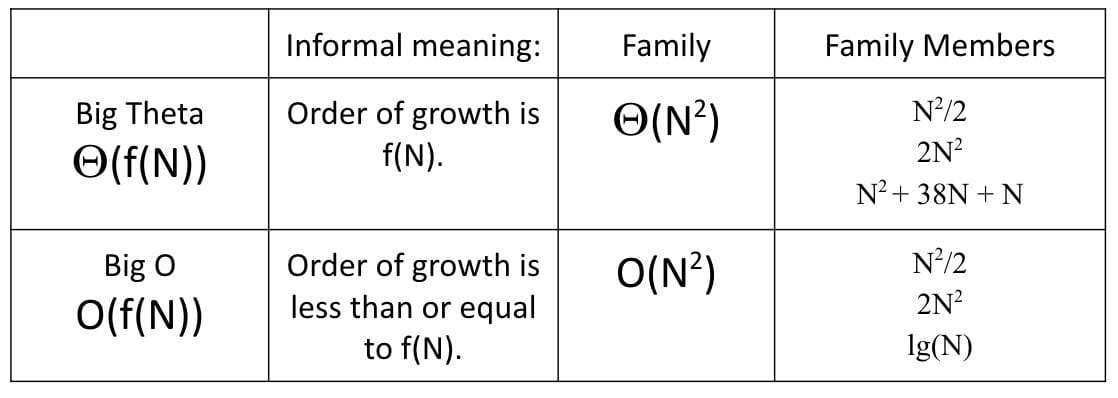Exercise:

Suppose we have a function bleepBlorp, and its runtime $R(N)$ has order of growth $\Theta(N^2)$. For Large $N$, if we run bleepBlorp on an input of size $N$, and an input size $10N$, we will have to wait roughly 100 times as long for the larger input.

True. It is the nature of quadratics.

If we run bleepBlorp on an input of size 1000, and an input of size 10000, we will have to wait roughly 100 times as long for the larger input.

False. 1000 may not be a large enough N to exhibit quadratic behavior.

### Some Practice

#### Loop Example 2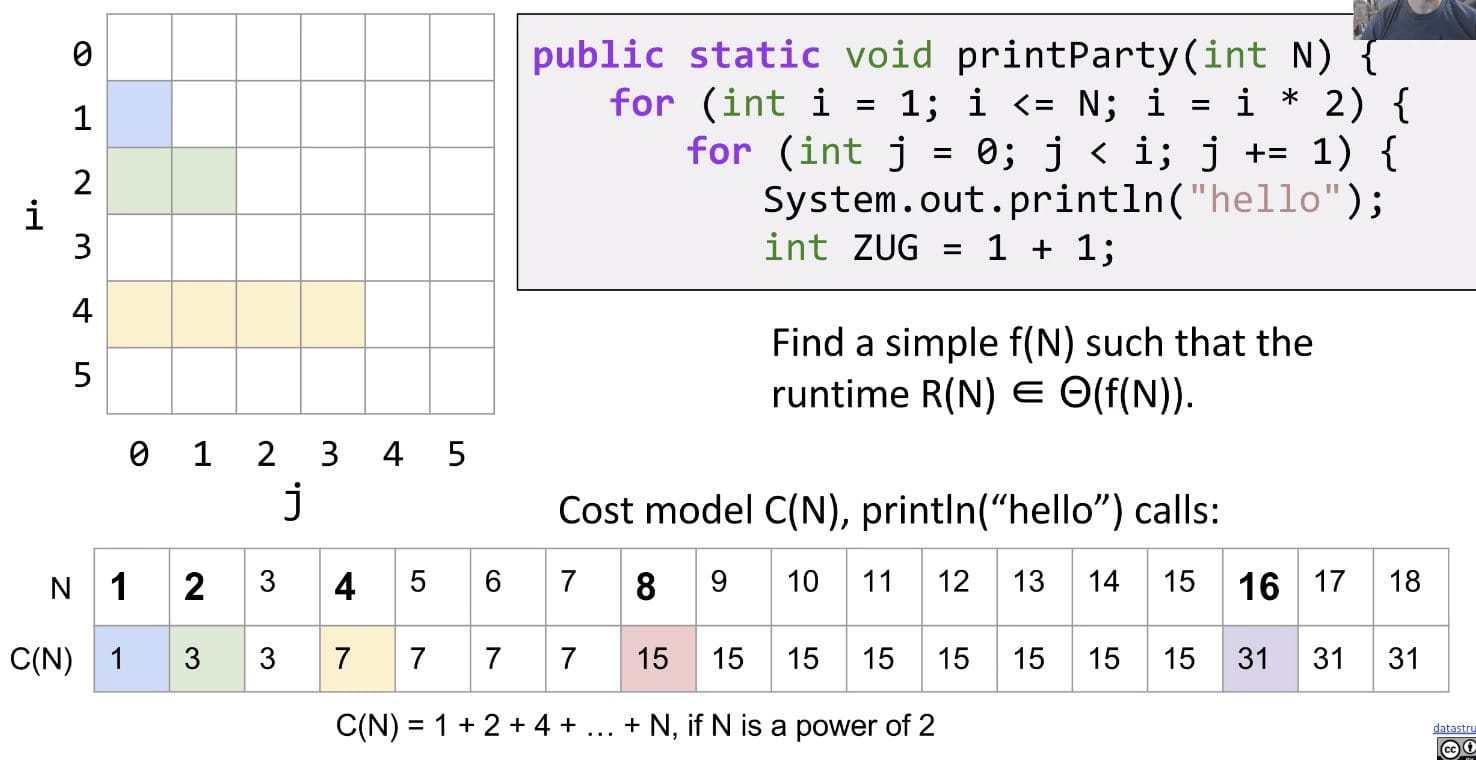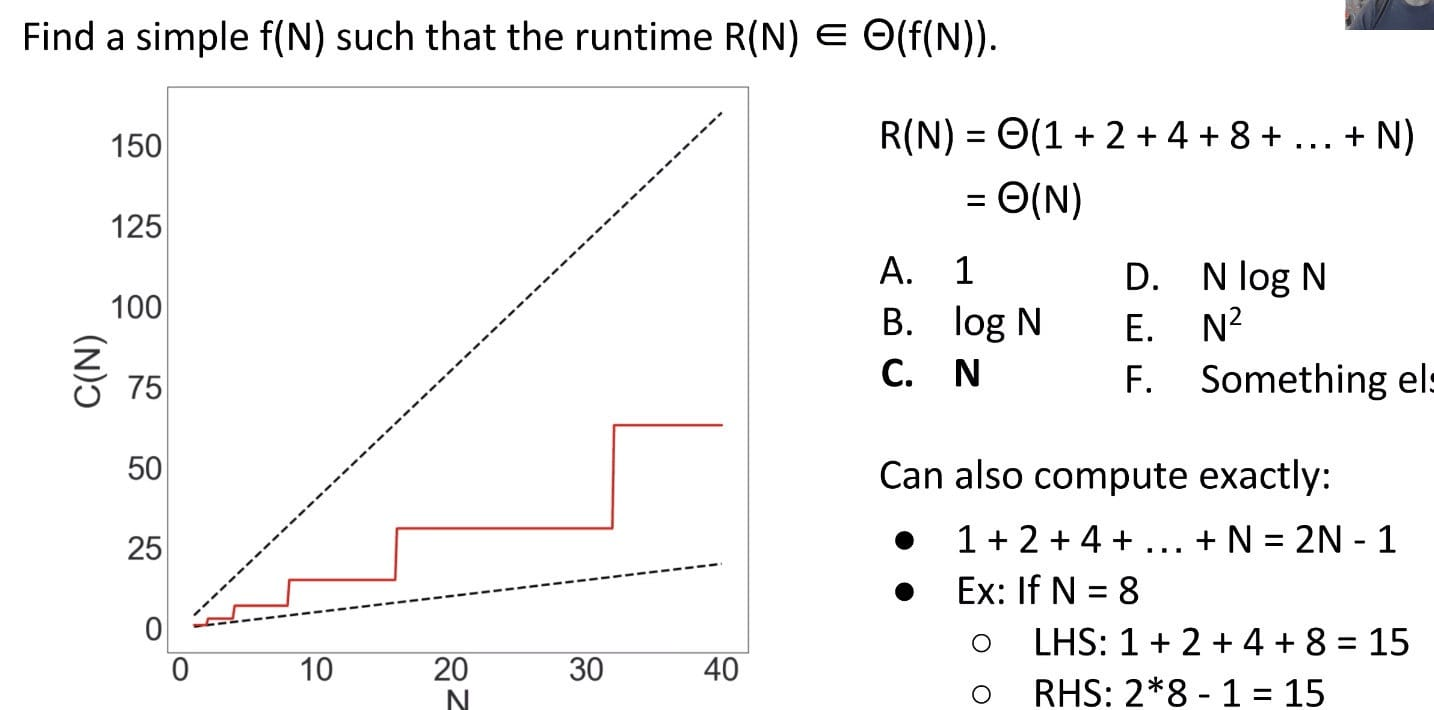Since the red line is bounded by two linear lines (4N and N), the runtime must also be linear!

$2^M = N, M = lgN$
$C(N) = 2^{(lgN + 1)} - 1 = 2^{(lgN + lg2)} - 1 = 2^{lg2N} - 1 = 2N - 1$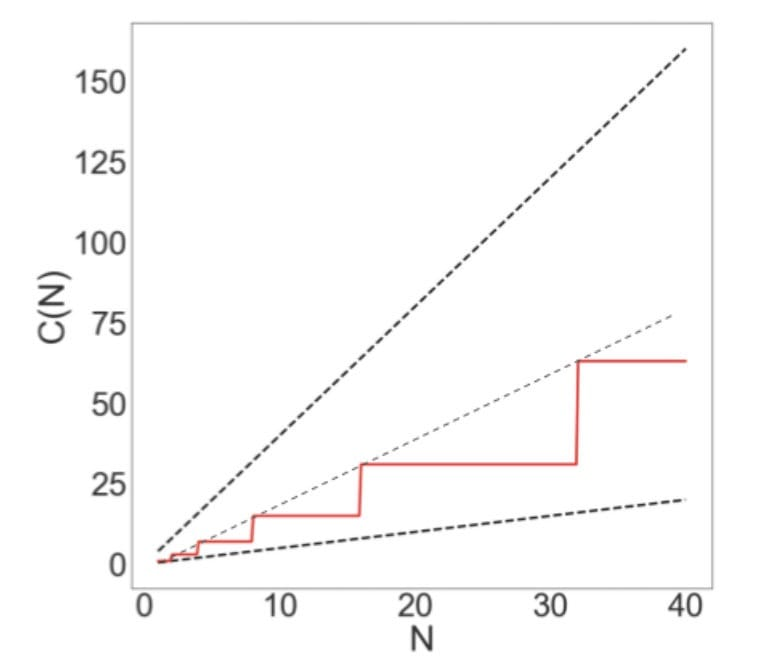It would be super convenient if all nested for loops were $N^2$. However, they’re not.

• There is no magic shortcut for these problems.
• Runtime analysis often requires careful thought.
• In programming, one little tiny statement in the middle of the code can cause the runtime to be completely different.
• In this class, there are basically two sums you need to know:
• Sum of First Natural Numbers: $1 + 2 + 3 + … + Q = \frac{Q(Q+1)}{2} = \Theta(Q^2)$
• Sum of First Powers of 2: $1 + 2 + 4 + 8 + … + Q = 2Q - 1 = \Theta(Q)$
• Strategies:
• Find exact sum
• Write out examples
• Draw pictures

#### Recursive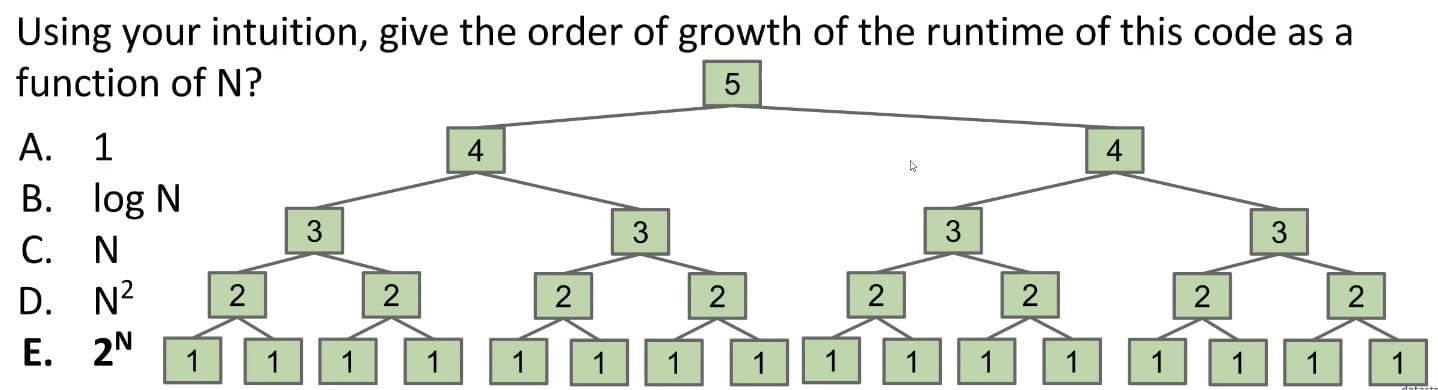Cost model: # of calls to f3.

2nd Approach (One math way):

• Consider the final term of sum? $2^{N-1}$
• So $C(N) = 1 + 2 + 4 + … + 2^{N-1}$
• According to sum of first powers of 2, $C(N) = 2 \times 2^{N - 1} - 1 = 2^N - 1$

3rd Approach (recurrence relation for C(N)):

• C(1) = 1
• C(N) = 2C(N-1) + 1 = 2(2C(N-2) + 1) + 1 = …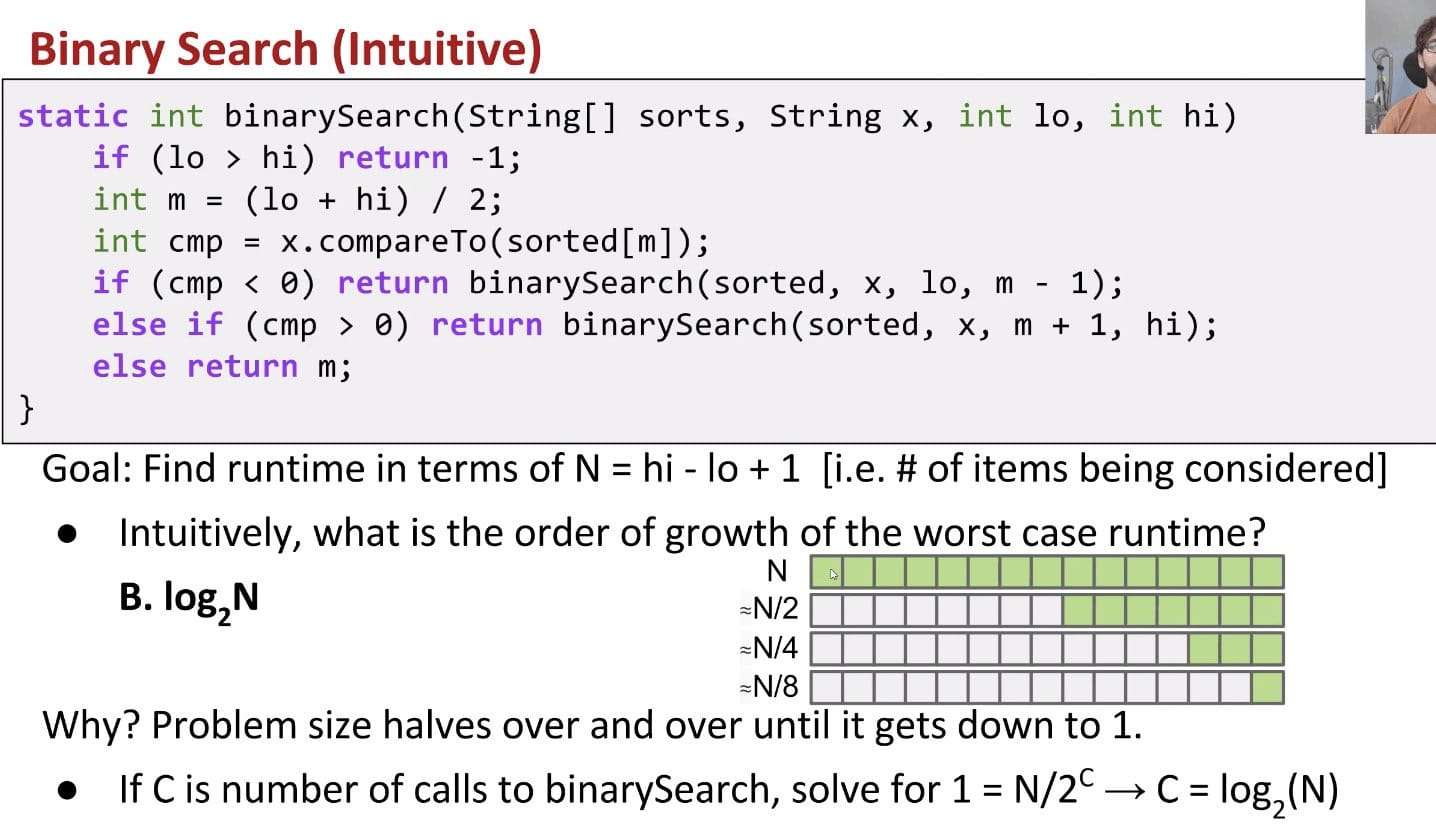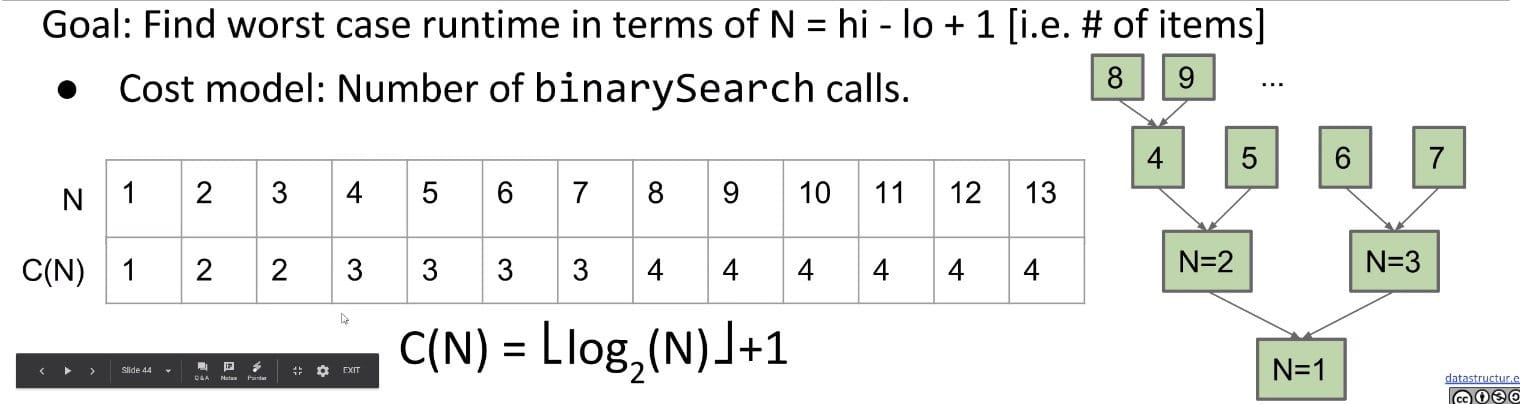These seems to support our intuition above of $\log_2 (n)$. We can see that the count seems to increase by one only when N hits a power of 2. But we can be more precise: $C(N) = floor(\log_2(N)) + 1$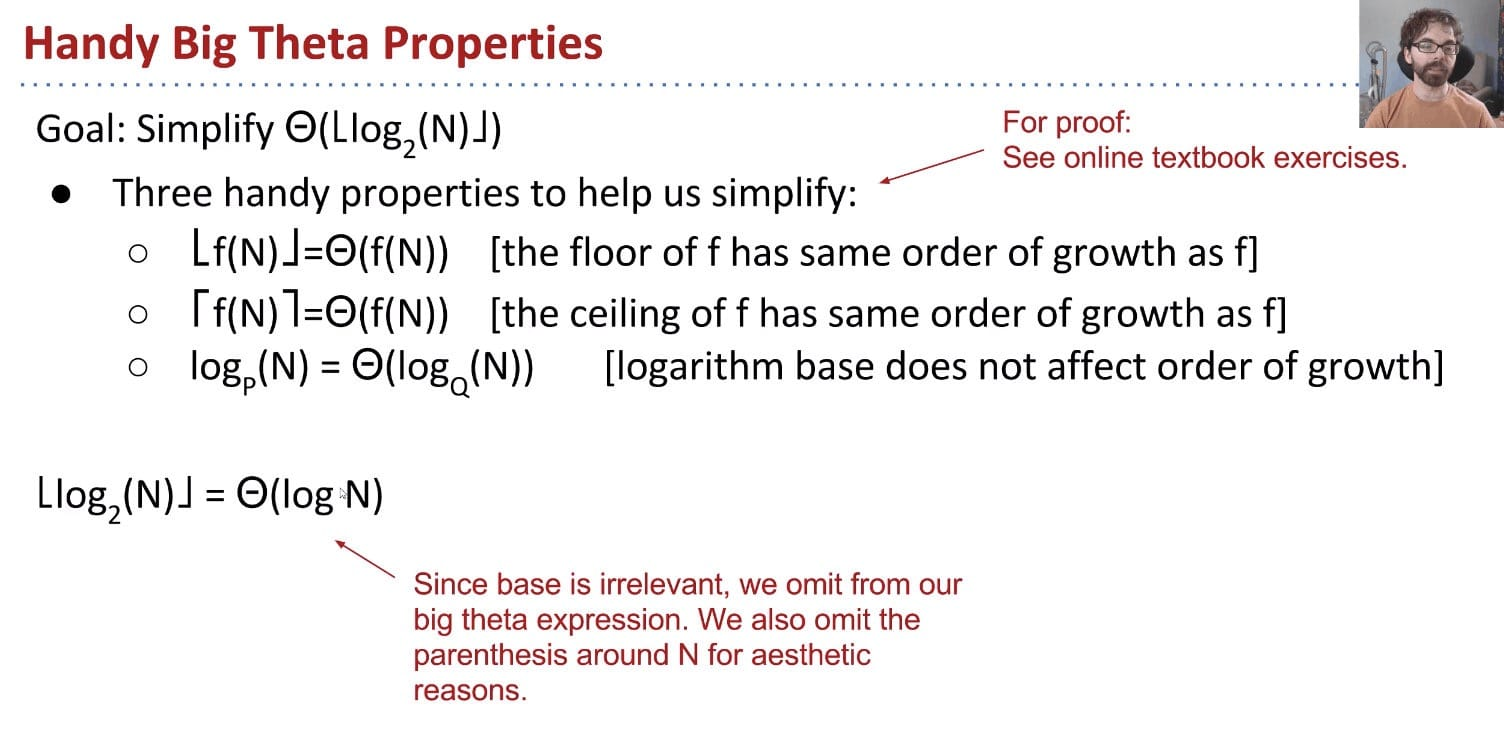The last one essentially states that for logarithmic runtimes, the base of the logarithm doesn't matter at all, because they are all equivalent in terms of Big-O (this can be seen by applying the logarithm change of base).

According to: $\log_b(a) = \frac{\log_x(a)}{\log_x(b)}$, we have: $\log_P(N) = \frac{\log_Q(N)}{\log_Q(P)}$.

Log time is super good! In practice, logarithmic time algorithms have almost constant runtimes. Even for incredibly huge datasets, practically equivalent to constant time.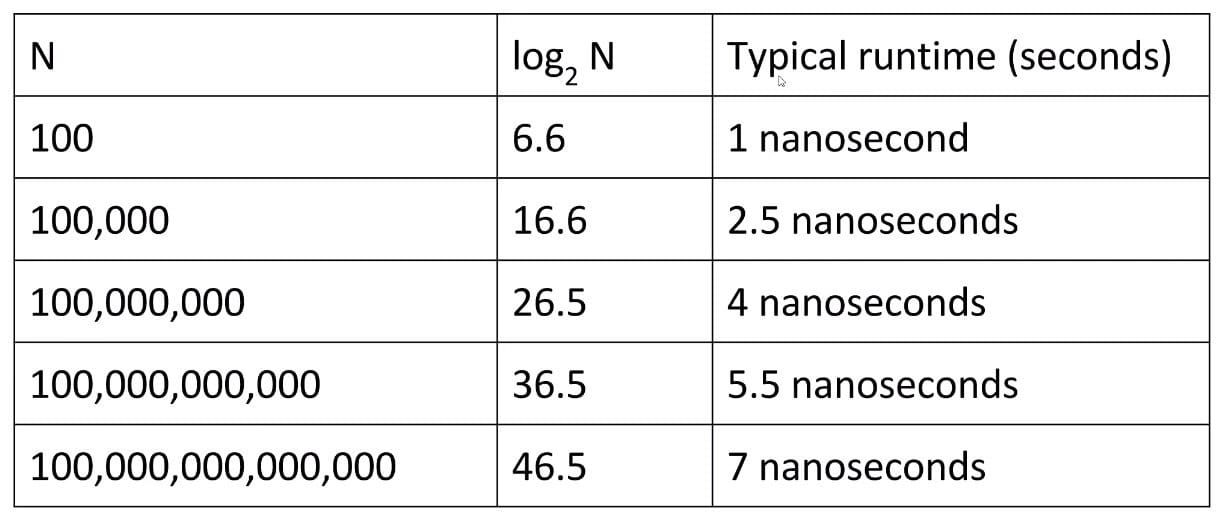#### Merge Sort

Selection sort:

• Find the smallest unfixed item, move it to the front, and ‘fix’ it.
• Sort the remaining unfixed items using selection sort.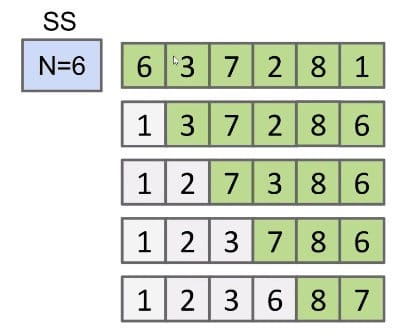Given that runtime is quadratic, for N = 64, we might say the runtime for selection sort is 2,048 arbitrary units of time (AU).

• N = 6 => ~36 AUs
• N = 64 => ~2048 AUs

Array Merging: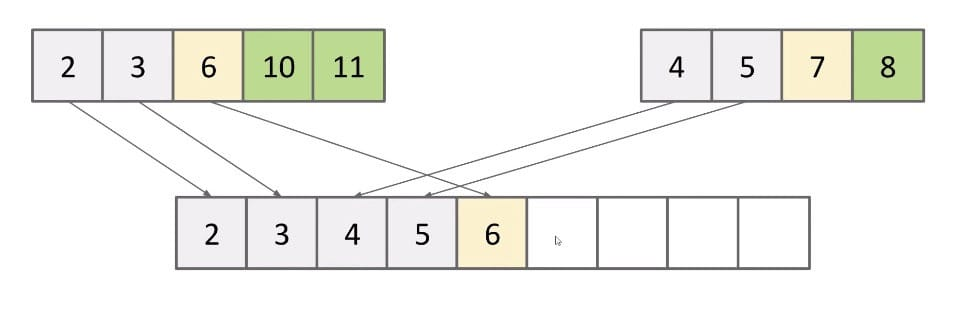The runtime is $\Theta(N)$. Use array writes as cost model.

Improvement

Merging can give us an improvement over vanilla selection sort:

• Selection sort the left half: $\Theta(N^2)$
• Selection sort the right half: $\Theta(N^2)$
• Merge the results: $\Theta(N)$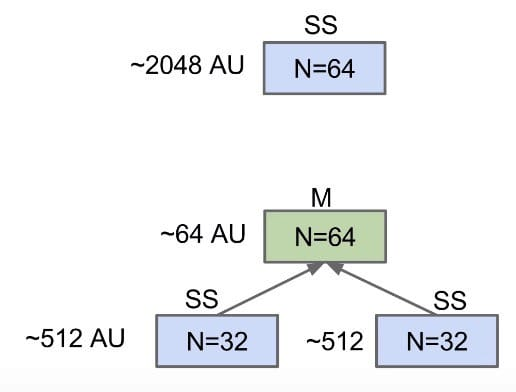Still $\Theta(N^2)$, but faster since $N + 2 \times (\frac{N}{2})^2 < N^2$

And we can go on doing it: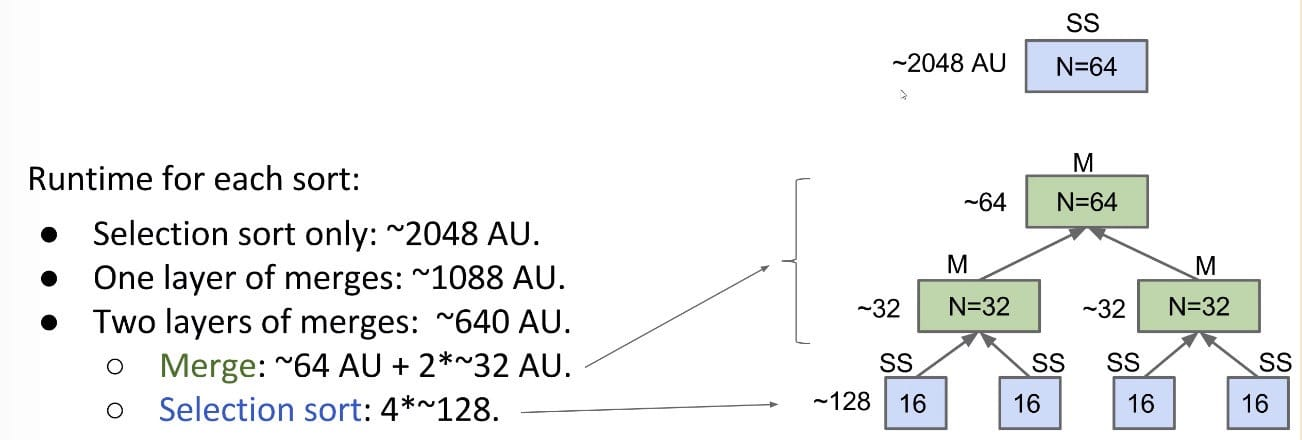Mergesort

Mergesort does merges all the way down (no selection sort):

1. If array is of size 1, return
2. Mergesort the left half
3. Mergesort the right half
4. Merge the results: $\Theta(N)$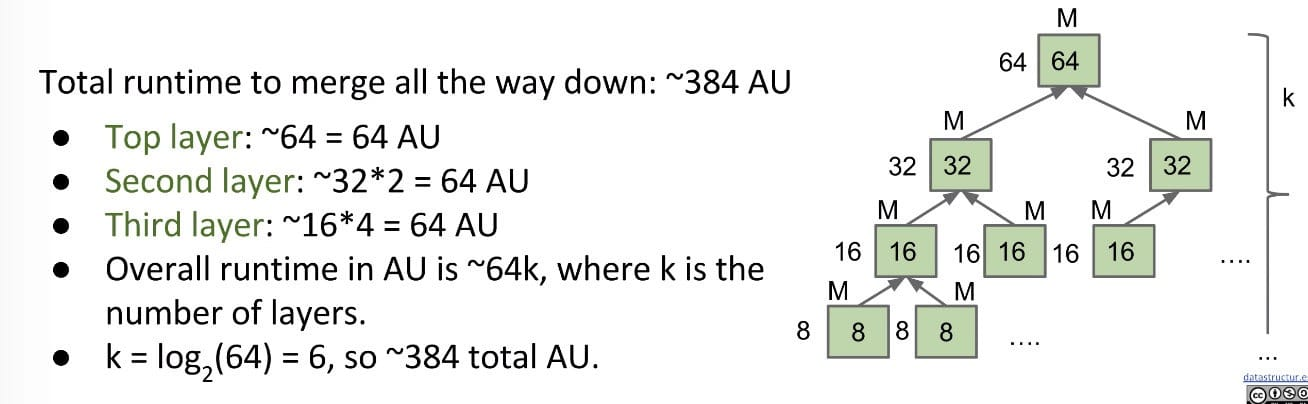For an array of size N, what is the worst case runtime of Mergesort?

For $N$, there will be $\log(N)$ layers, and each layer contains $N$ AUs. So in total there are $N\log(N)$ AUs.

Overview

$N\log N$ is basically as good as $N$, and is vastly better than $N^2$.

Going from $N\log N$ to $N$ is nice, but not a radical change.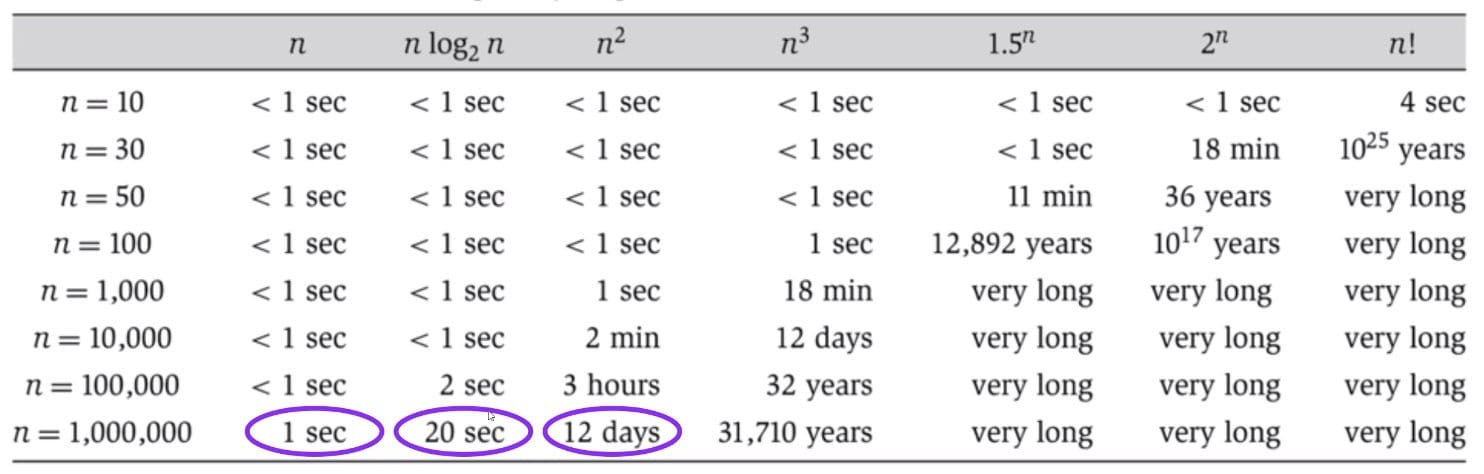### Big O vs. Big Theta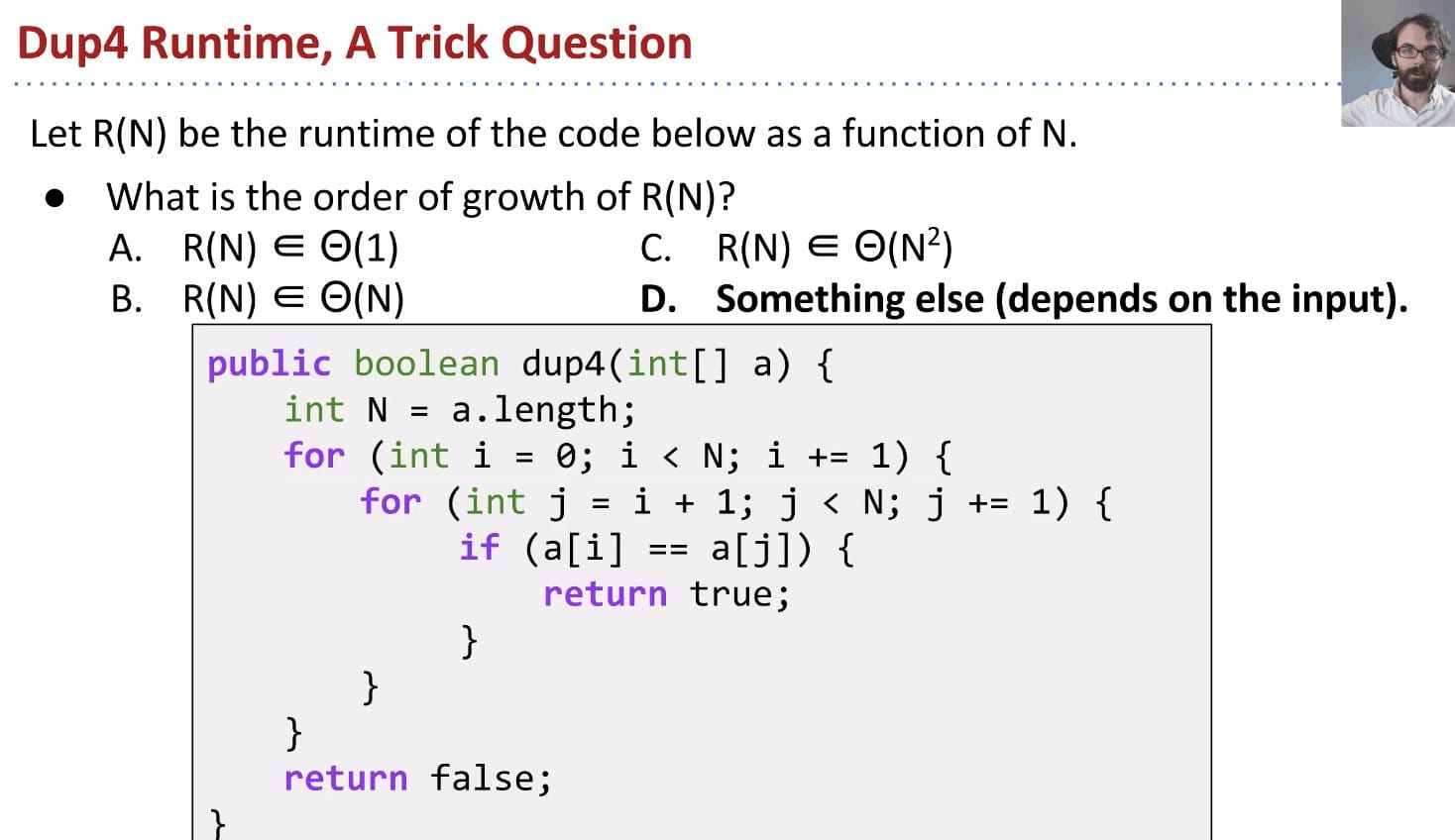• The best case runtime: $\Theta(1)$
• The worst case runtime: $\Theta(N^2)$
• Or we can just use big O and avoid qualifying our statement at all, i.e. $O(N^2)$.

The Limitations of Big Theta

Big Theta expresses exact order of growth for runtime in terms of $N$. If runtime depends on more factors than just $N$, may need different Big Theta for every interesting condition.

Big O Abuse

Which statement gives you more information about the runtime of a piece of code?

1. The worst case runtime is $\Theta(N^2)$ (this one)
2. The runtime is $O(N^2)$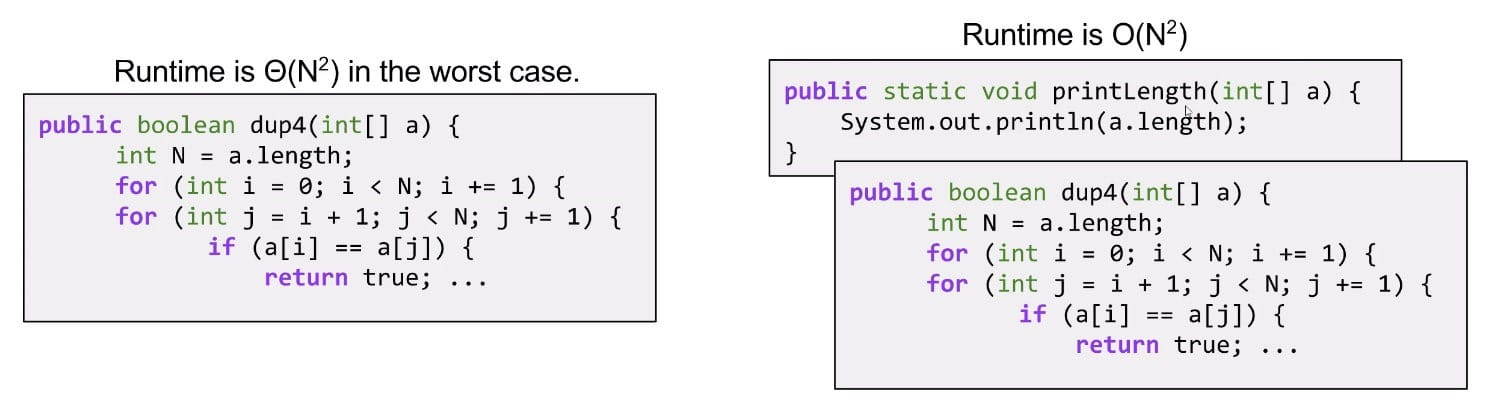Big Theta vs. Big O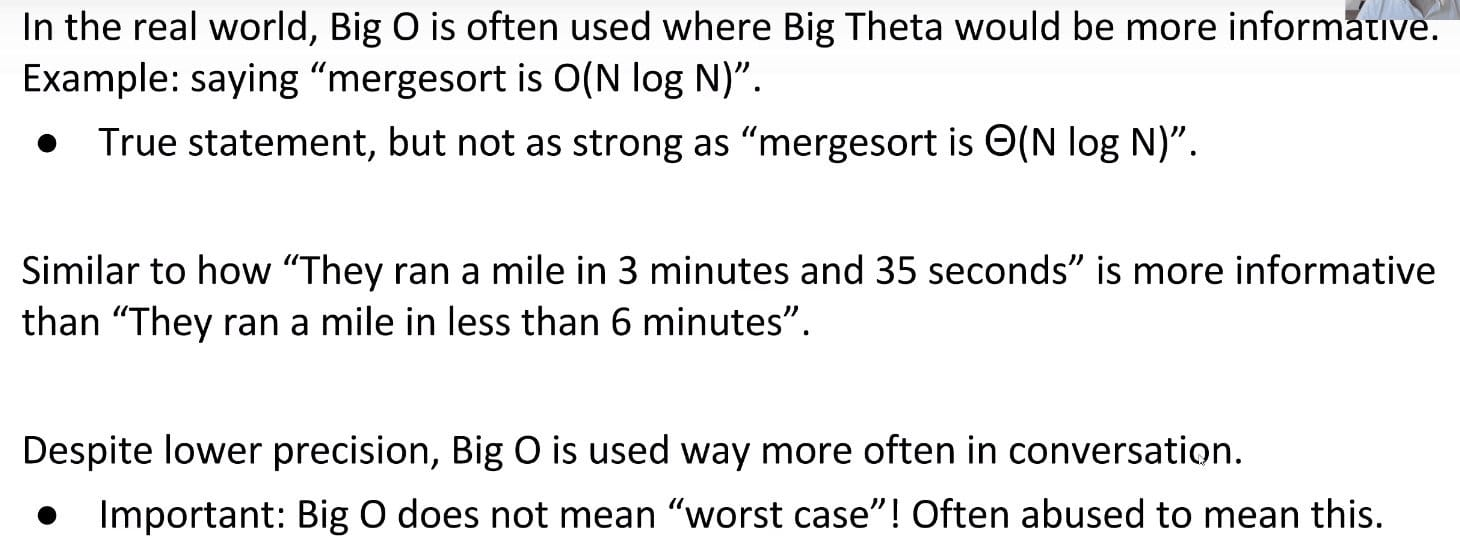Note: Big O is NOT the same as “worst case”. But it is often used as such.

Big O is still a useful idea:

• Allows us to make simple blanket statements without case qualifications, e.g. can just say “binary search is $O(log N)$” instead of “binary search is $\Theta(log N)$ in the worst case”.
• Sometimes don’t know the exact runtime, so use $O$ to give an upper bound.
• Example: Runtime for finding shortest route that goes to all world cities is $O(2^N)^*$. There might be a faster way, but nobody knows one yet.
• Easier to write proofs for Big O than Big Theta.

### Big Omega - $\Omega(N)$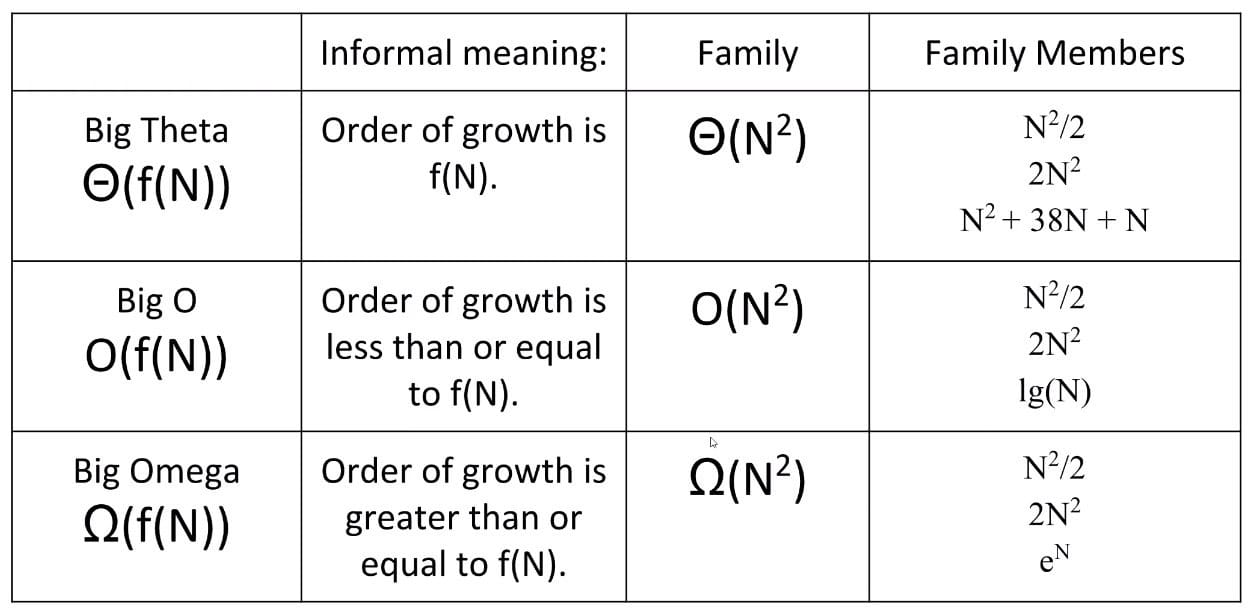Two common uses for Big Omega:

• Very careful proofs of Big Theta runtime.
• If $R(N) = O(f(N))$ and $R(N) = \Omega(f(N))$, then $R(N) = \Theta(f(N))$.
• Sometimes it’s easier to show $O$ and $\Omega$ separately.
• Providing lower bounds for the hardness of a problem.
• Example: The time to find whether an array has duplicates is $\Omega(N)$ in the worst case (have to actually look at every item).

Note: Big Omega does NOT mean best case.

### Amortized Analysis

It provides a way to prove the average cost of operations.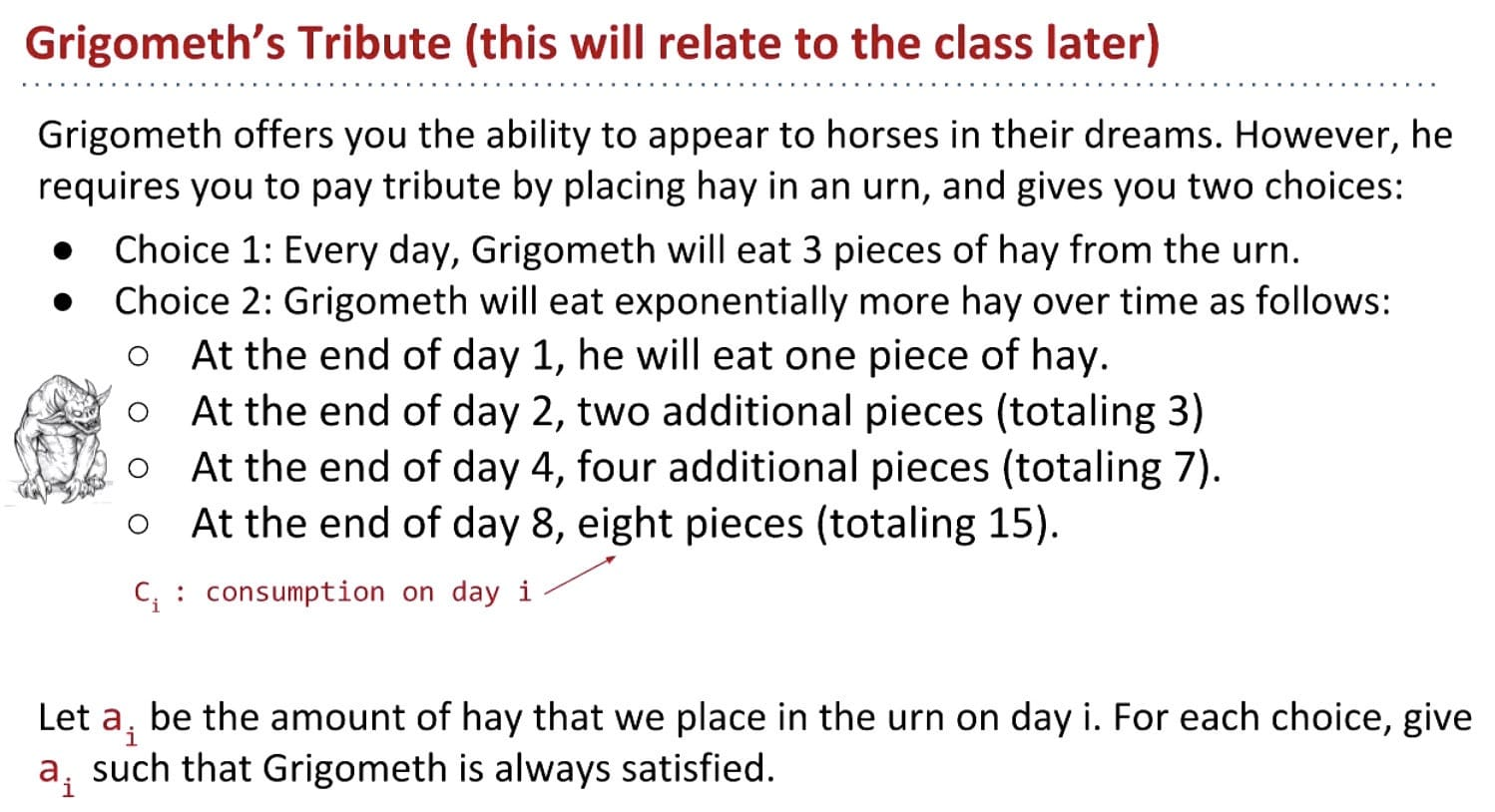This is related to resizing in AList.

Geometric Resizing

It turns out, Grigometh’s hay dilemma is very similar to AList resizing from early in the course.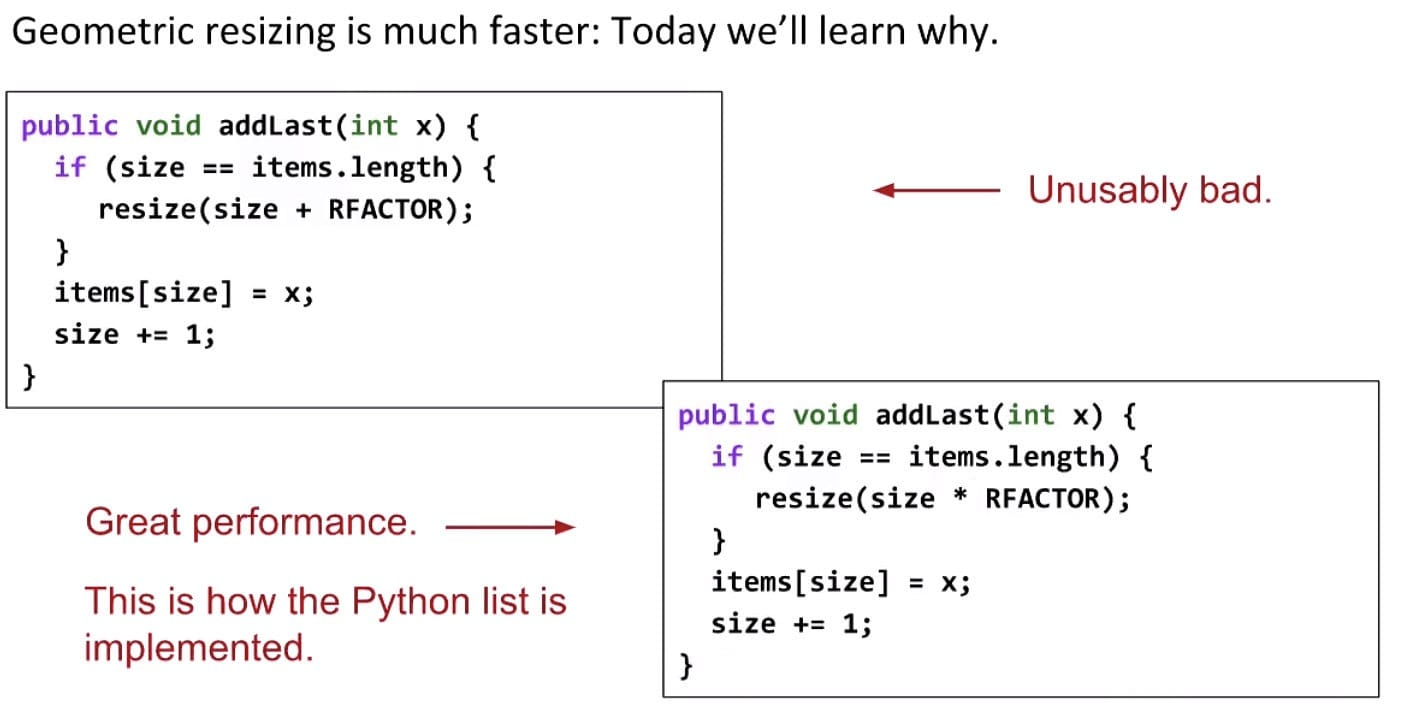As for the second implementation, most add operations take $\Theta(1)$ time, but some are very expensive, and linear to the current size. However, if we average out the cost of expensive adds with resize over all the adds that are cheap, it turns out that on average, the runtime of add is $\Theta(1)$.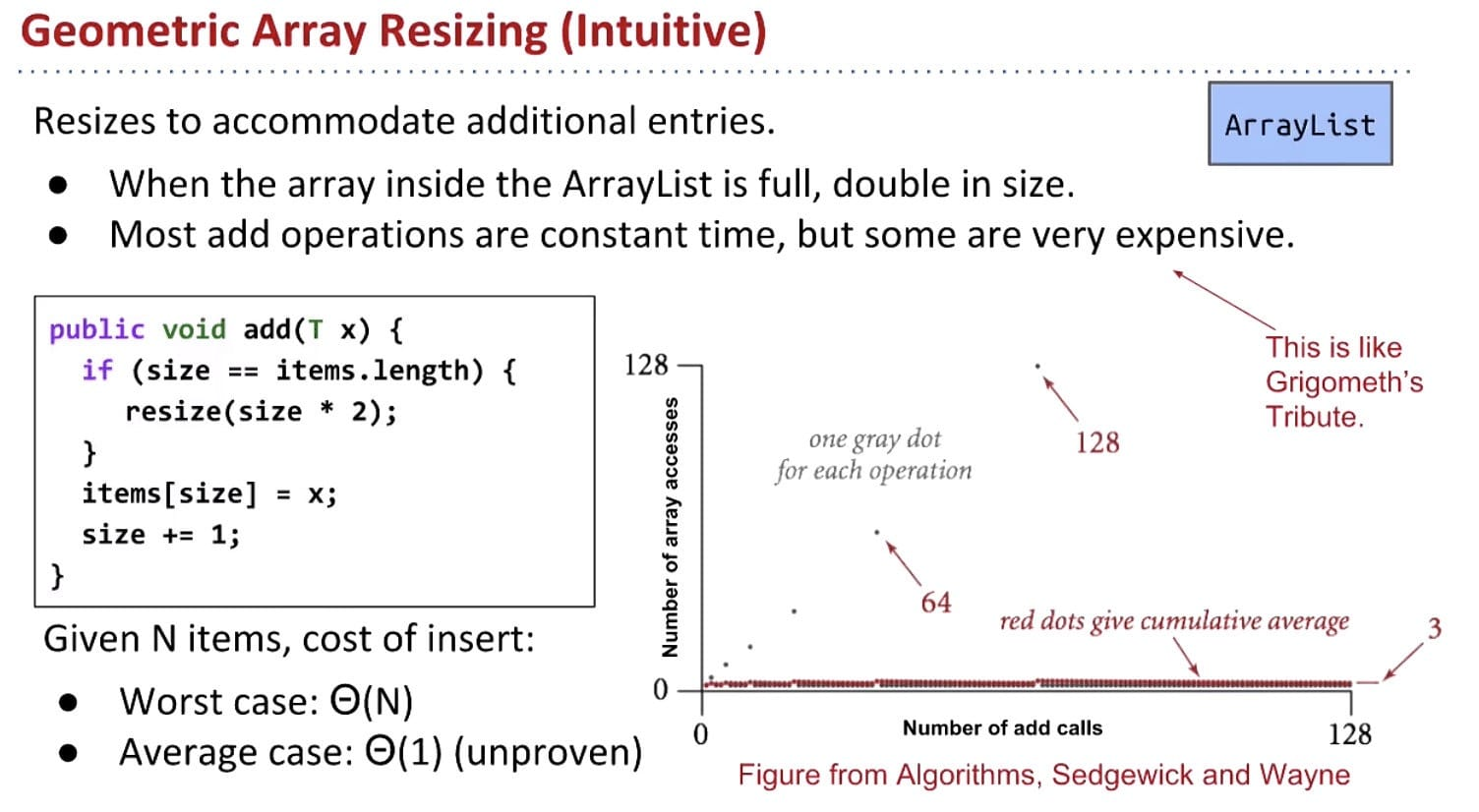A more rigorous examination of amortized analysis is done here, in three steps:

• Pick a cost model (like in regular runtime analysis)
• Compute the average cost of the i-th operation
• Show that this average (amortized) cost is bounded by a constant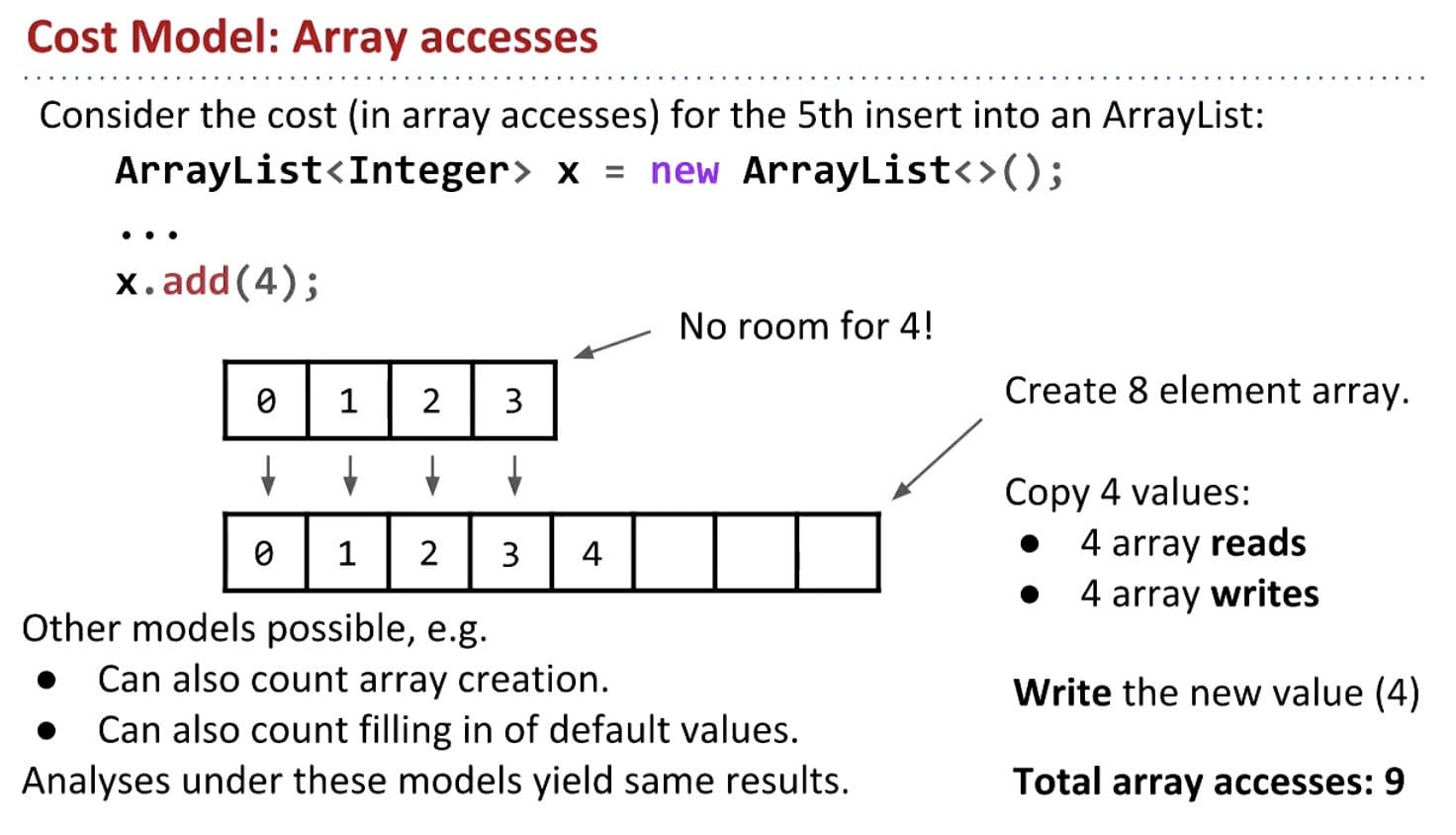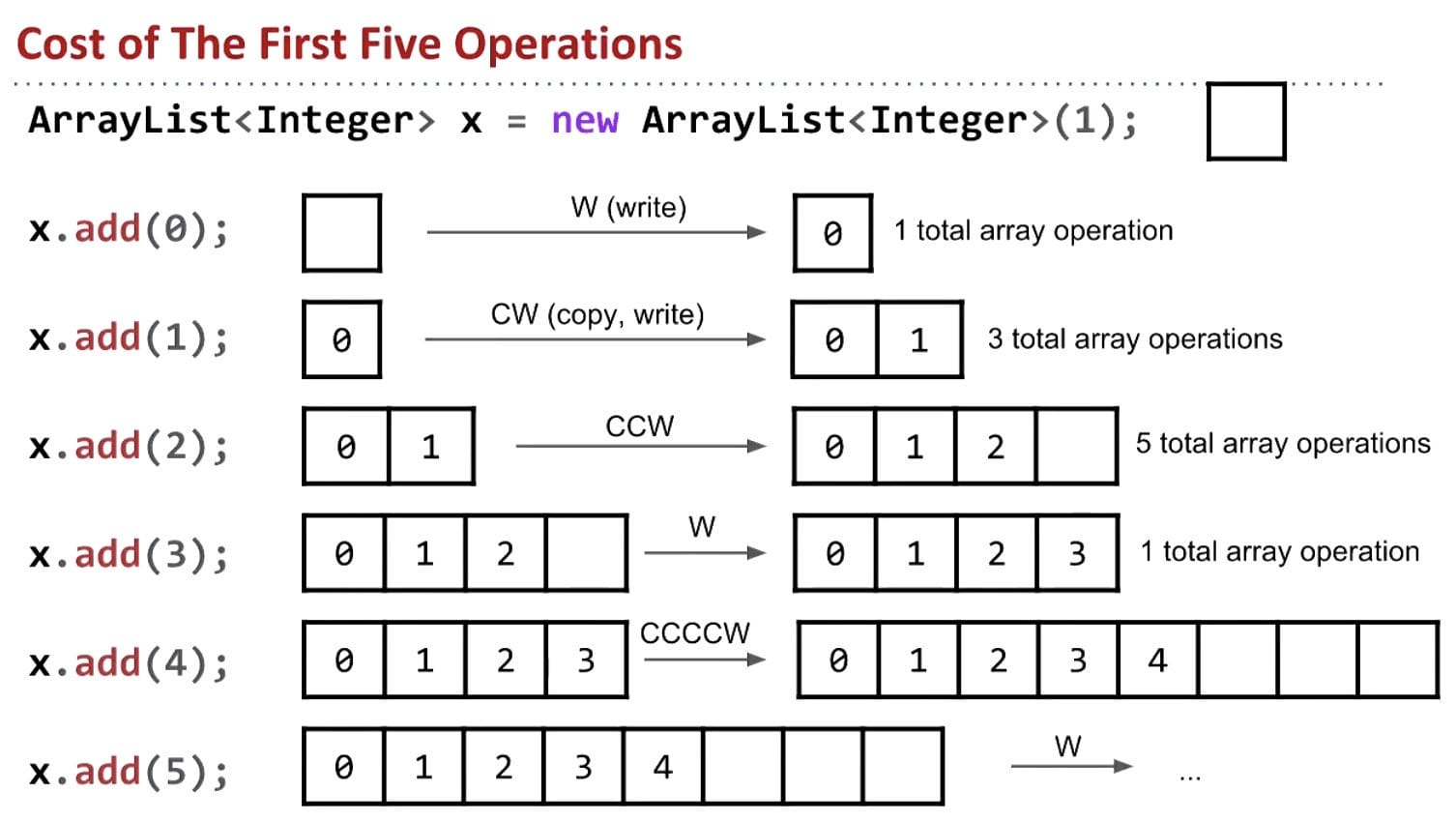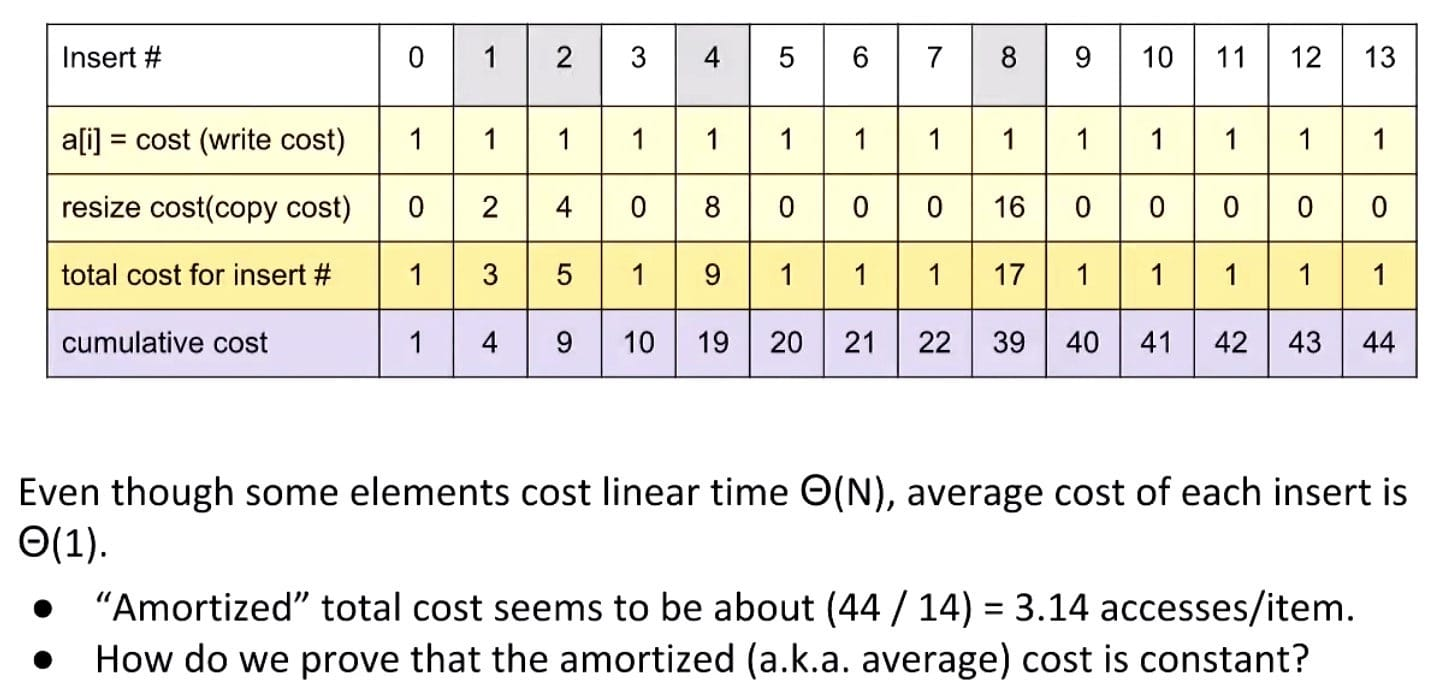But for the first 8 adds, the average cost is 39/8 = 4.875 per add. It seems like it might be bounded by 5. But just by looking at the first 13 adds, we cannot be completely sure.

The Idea of Potential

We’ll now introduce the idea of potential to aid us in solving this amortization mystery.

If we choose $a_i$ such that $\Phi_i$ is never negative and $a_i$ is constant for all $i$, then the amortized cost is an upper bound on the true cost.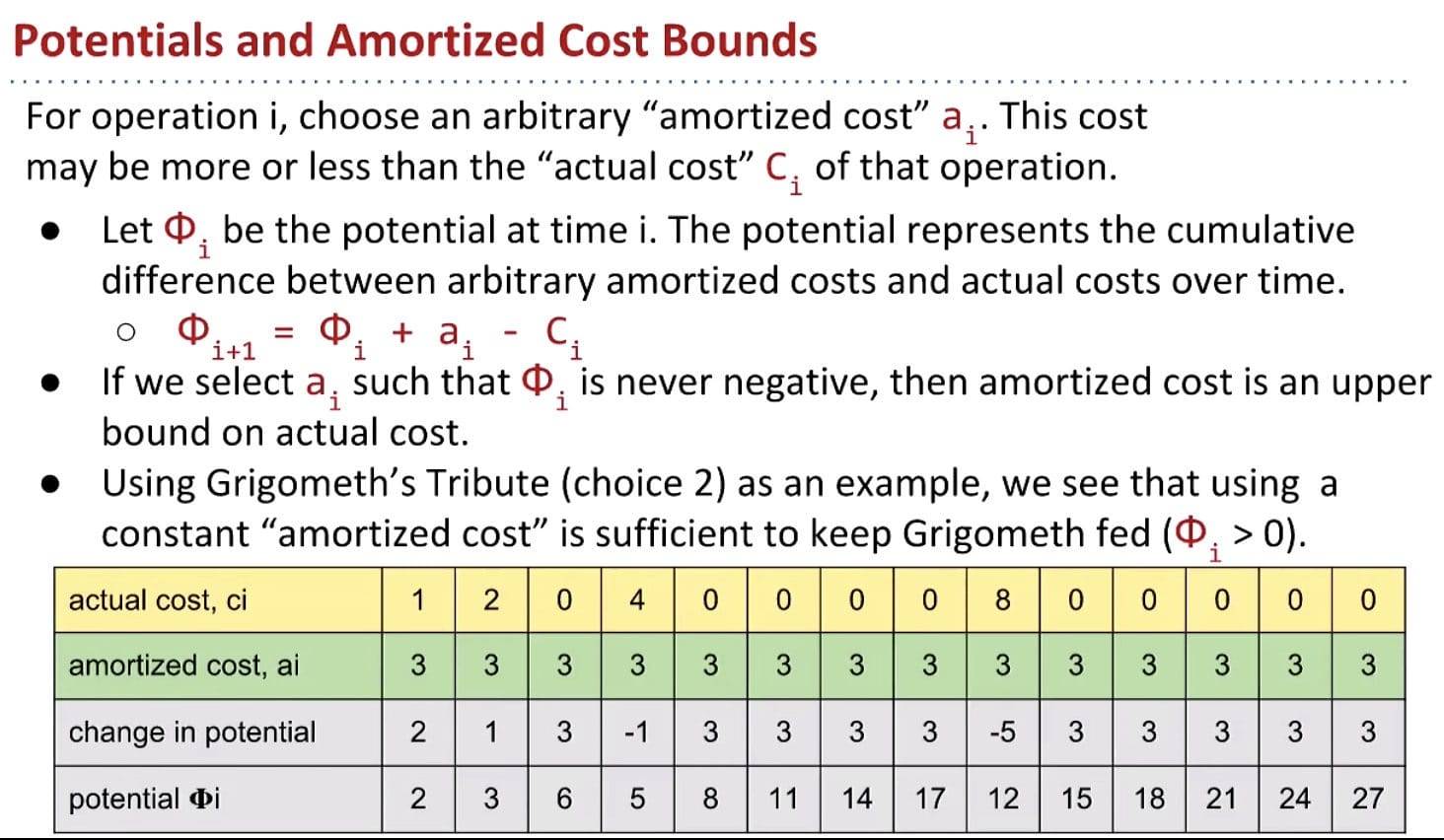Now back to ArrayList resizing

Goals for ArrayList: $a_i \in \Theta(1)$ and $\Phi_i \geq 0$ for all $i$.

Key idea: Choosing $a_i$ so that $\Phi_i$ stays positive.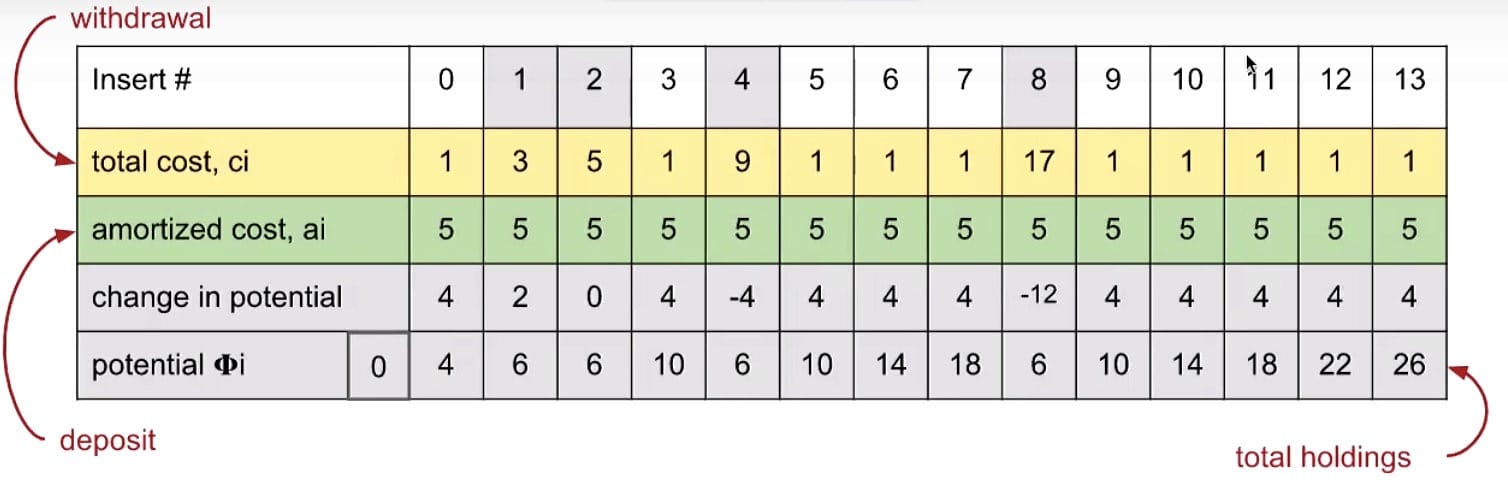Finally, we’ve shown that ArrayList add operations are indeed amortized constant time. Geometric resizing (multiplying by the RFACTOR) leads to good list performance.

## Ex (Discussion 7)

• Any exponential dominates any polynomial
• Any polynomial dominates any logarithm

Order: $\Theta(1) < \Theta(\log{n}) < \Theta(n) < \Theta(n\log{n}) < \Theta(n^2) < \Theta(n^2 \log{n}) < \Theta(n^3) < \Theta(2^n) < \Theta(n!) < \Theta(n^n)$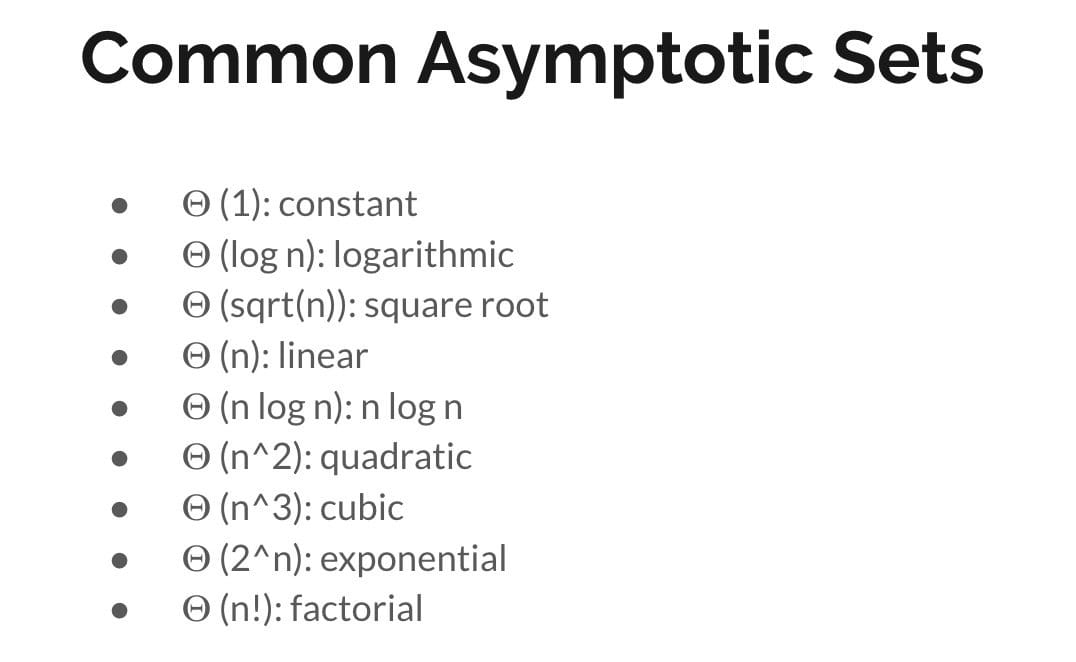### Problem 2a

Runtime: $O(M + N)$ (Base case: $N$)

### Problem 2b

Worst: $\Theta(N \log{N} + N^2) = \Theta(N^2)$
Best: $\Theta(N \log N + 0) = \Theta(N \log N)$

### Problem 2c

Worst: $\Theta(NM)$
Best: $\Theta(N \log M)$

### Problem 3

Note: the array is sorted!

Worst: $\Theta(N^2)$
Best: $\Theta(1)$

Worst: $\Theta(N)$
Best: $\Theta(1)$

## Ex (Guide)

### C Level

What would the runtime of modified_fib be. Assume that values is an array of size n. If a value in an int array is not initialized to a number, it is automatically set to 0.

Cost Model: array writes

$N$ 0 1 2 3 4 5 6
$C(N)$ 0 0 3 5 9 15 25

$C(N) = C(N - 1) + C(N - 2)$
$C(N) = (\frac{1 + \sqrt{5}}{2})^N + (\frac{1 - \sqrt{5}}{2})^N$
$T(N) = O((\frac{1 + \sqrt{5}}{2})^N + (\frac{1 - \sqrt{5}}{2})^N) = O((\frac{1 + \sqrt{5}}{2})^N) = O(1.6180^N)$

link

### B Level

• Find the runtime of running print_fib with for arbitrary large n.

Cost model: # of calls to fib()

$C(N) = 1.618^0 + 1.618^1 + 1.618^2 + 1.618^3 + … + 1.618^N = 1 + \frac{1.618 (1 - 1.618^N)}{1 - 1.618} \in \Theta(1.618^N)$

$\Theta(f(N)) = \Theta(1.618^N)$

• Do the problem again, but change the body of the for loop in print_fib to be:

$O(f(N)) = O(N \times 1.618^N)$

• Find the runtime of this function:

Cost Model: println
$\Theta(N^2 + N^3) = \Theta(N^3)$

• Find the runtime of this function:

Cost Model: println

First, let’s just assume there is only one call grigobreath(N * 1/2), and the number of operations is $C_1(N)$.

According to the table, $C_1(N) = 2N - 1 \in \Theta(N)$ (Assume operation takes constant time).

As for two C2, since N gets smaller faster, # of operations in C2 is less than the # in C1.

So, $C(N) \in \Theta(N)$.

$N$ 0 1 2 3 4 5 6 7 8
$C_1(N)$ 0 1 3 4 7 8 10 11 15

### Problem 8

From: Spring 2018 Midterm #2

a)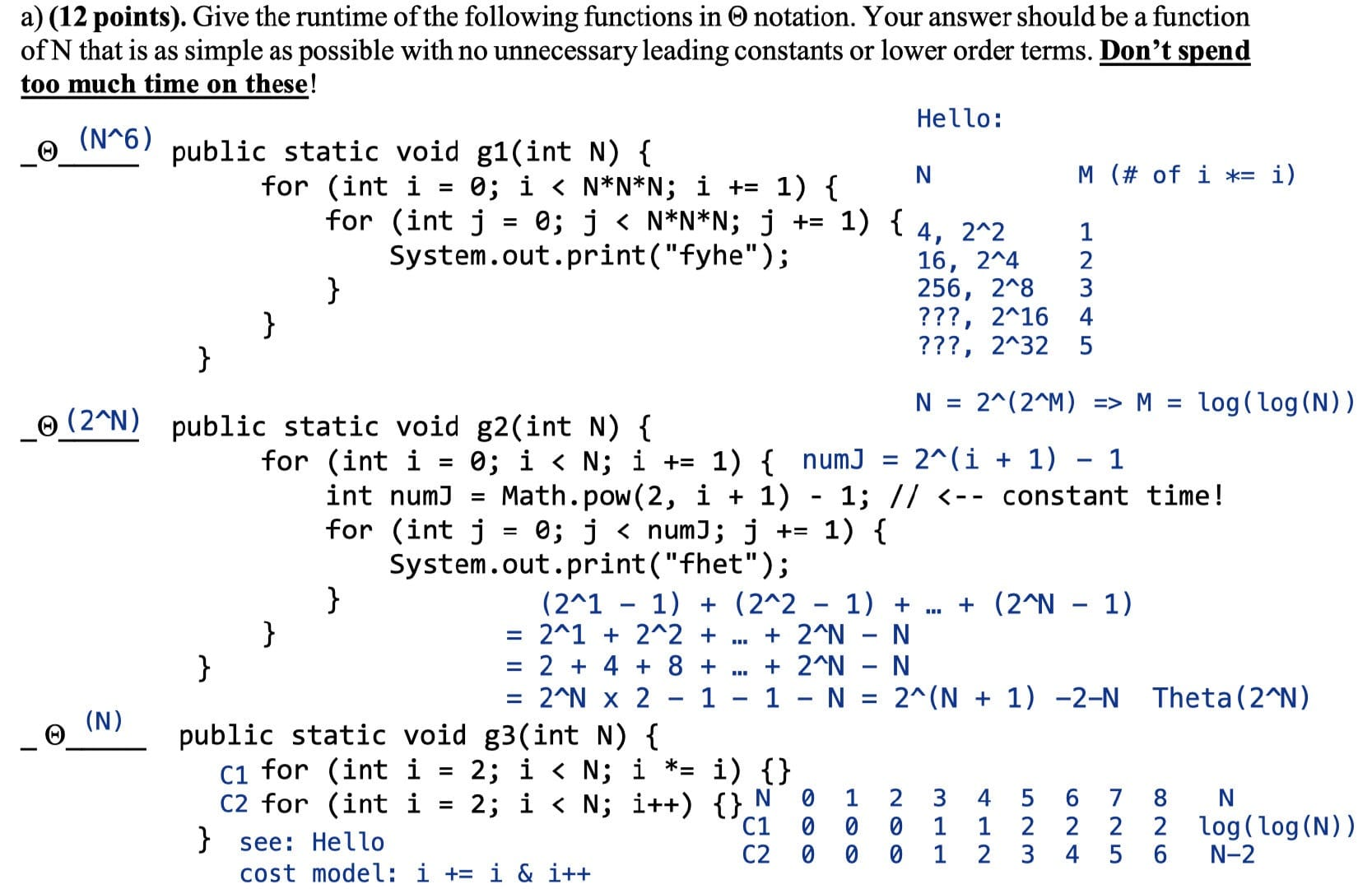Another way to think about the 3rd problem is that the first for-loop is less than $N$ and the second is approximately $N$, so it’s not necessary to compute C1.

b) & c)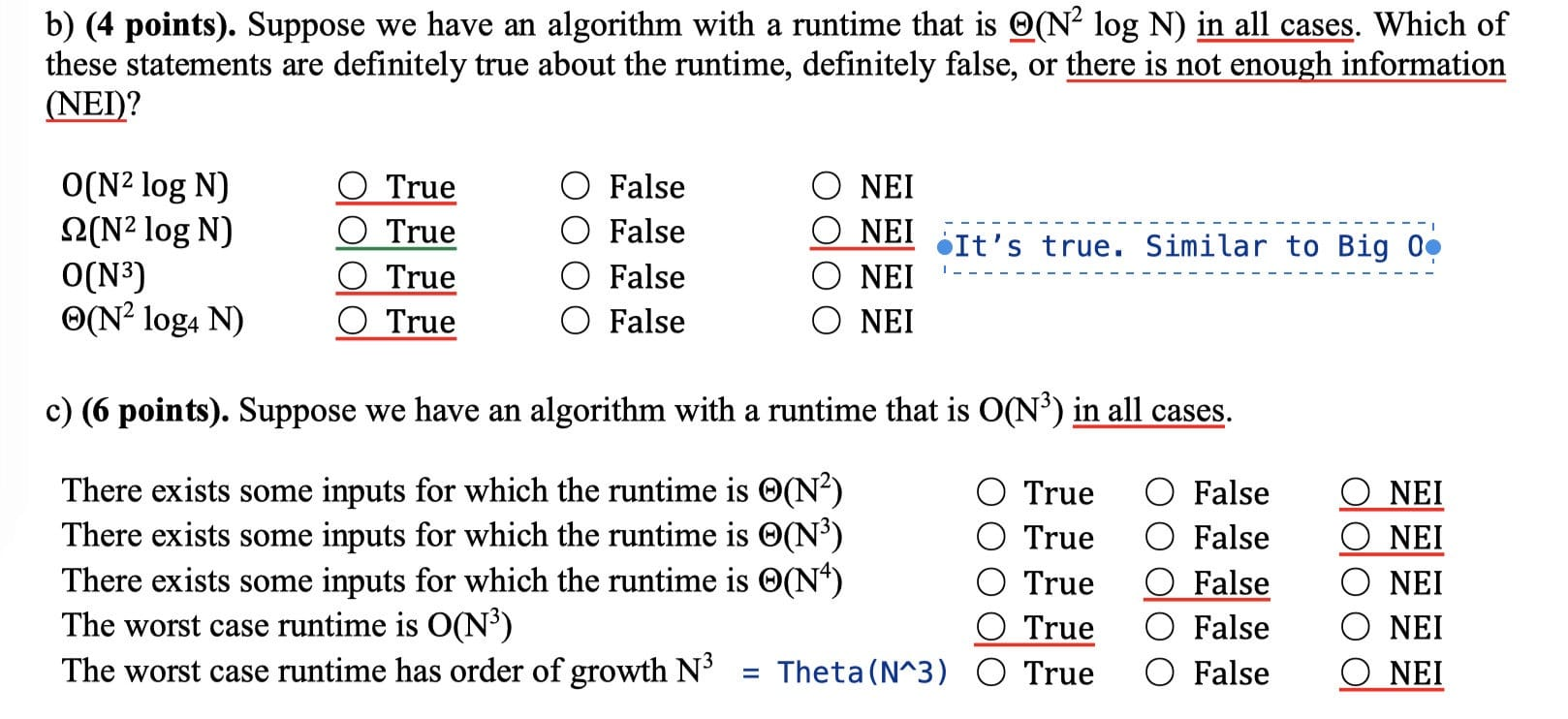d)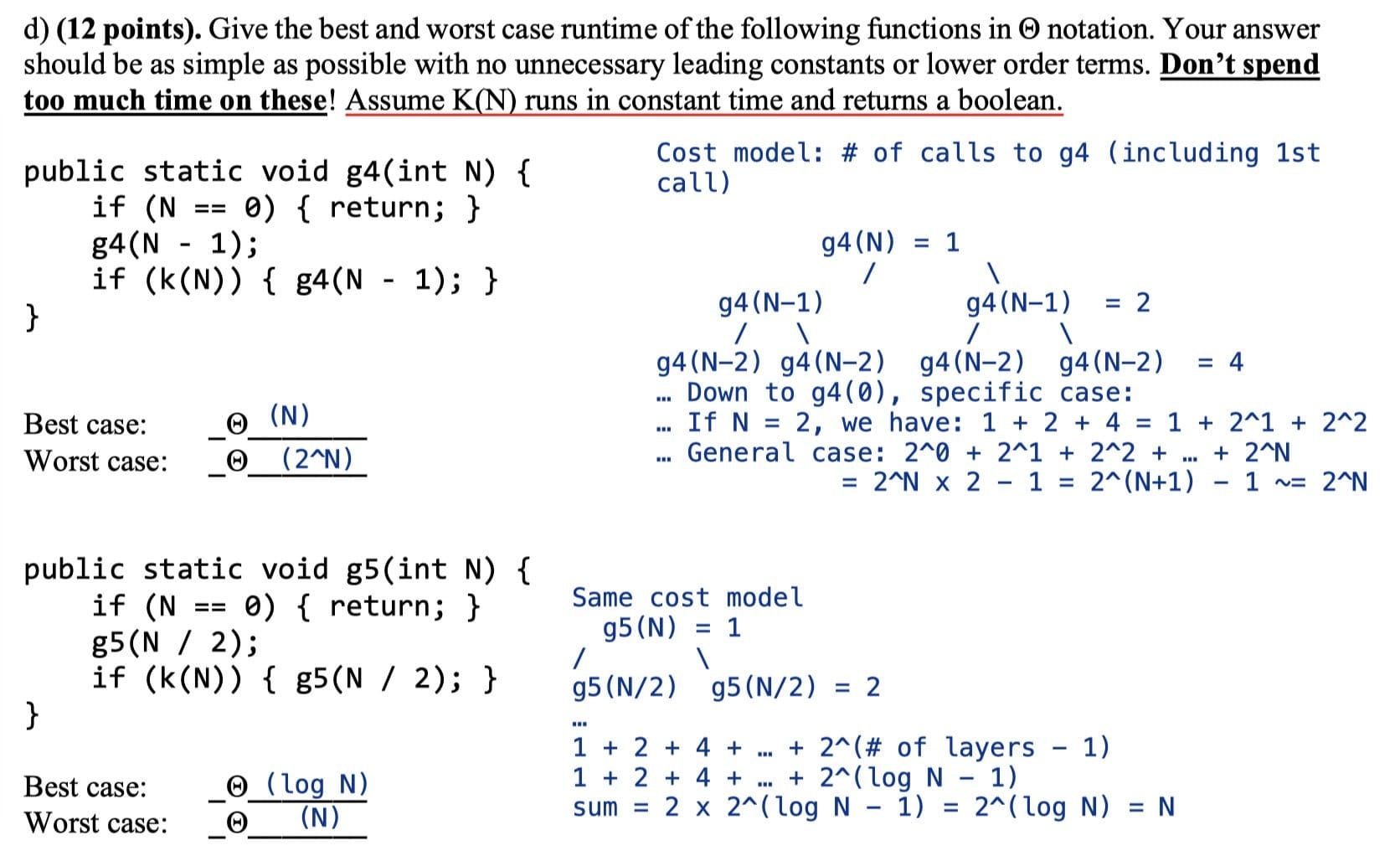e) & f)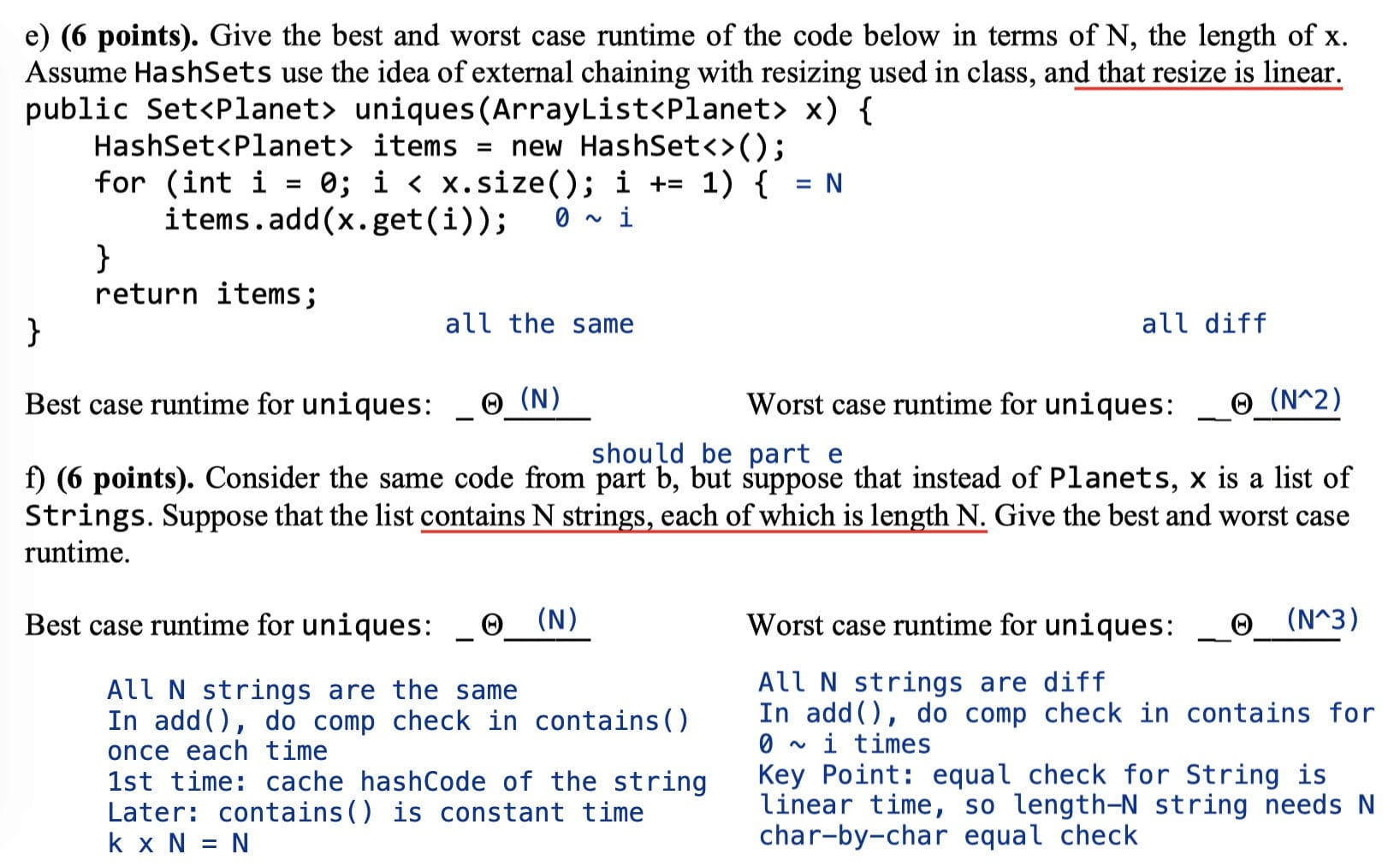### Problem 4

From: Spring 2017 Midterm #2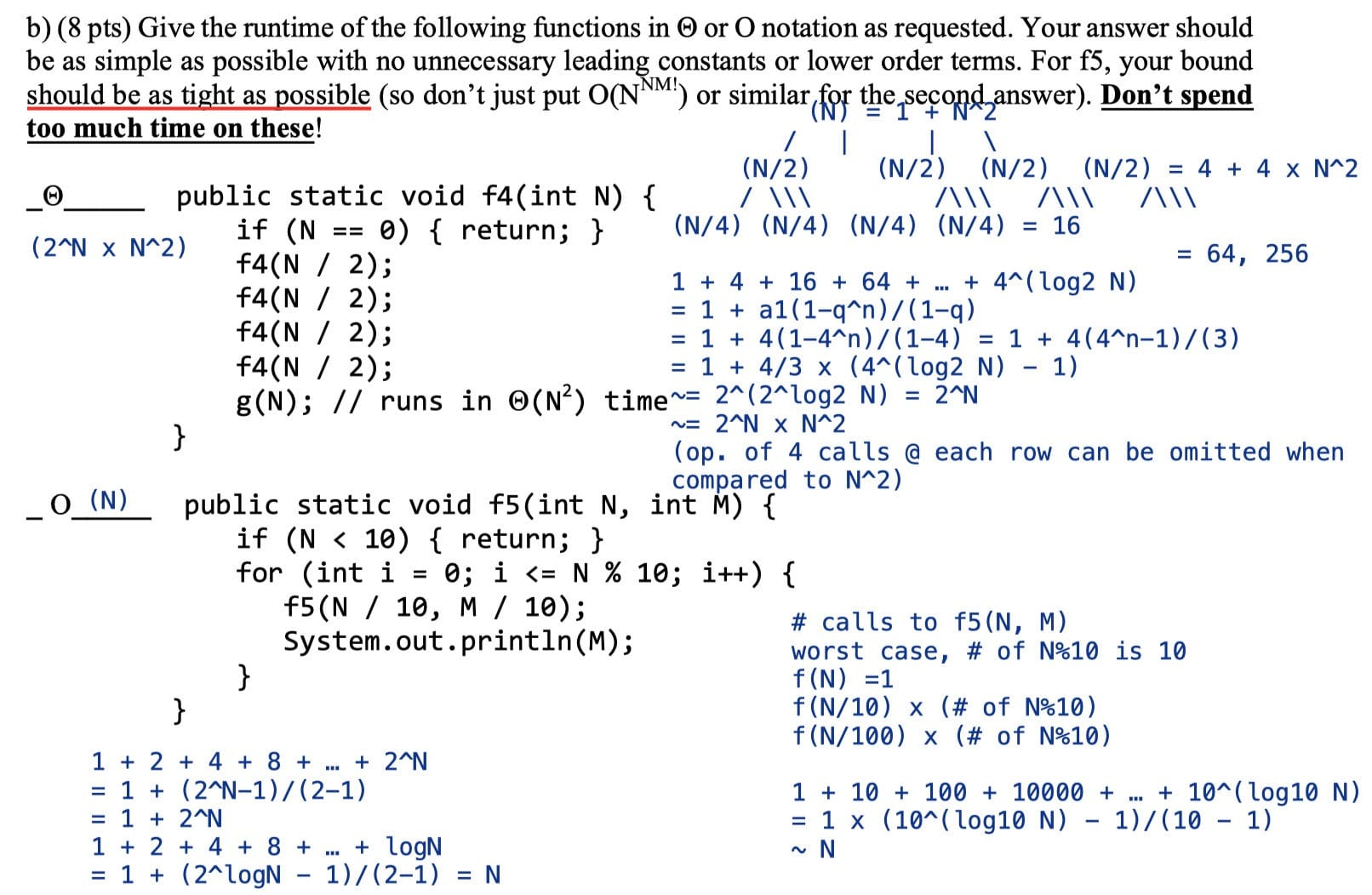f4: $4^0 \times N^2 + 4^1 \times (N-1)^2 + 4^2 \times (N-2)^2 + \dots + 4^N \times 1^2$

Official Answer: $\Theta(N^2 \log N)$

## Ex (Discussion 7, Spring 19)

Space Jam 2 - Give the worst case and best case runtime in $\Theta(\cdot)$ notation.

### andslam

$N + \frac{N}{2} + \frac{N}{4} + \frac{N}{8} + … + 1 = N \times (1 + \frac{1}{2} + \frac{1}{4} + \frac{1}{8} + … + \frac{1}{N}) \sim 2N \in \Theta(N)$

Worst case: $\Theta(N)$
Best case: $\Theta(N)$

In both cases the runtime is $\sum^{\log(N)}_{i=0} \frac{N}{2^i} \leq N \sum^{\log(\infty)}_{i=0} \frac{1}{2^i} = 2N$. One potentially tricky portion is that $\sum^{\log(n)}_{i=0}\frac{1}{2^i}$ is at most 2 because the geometric sum as it goes to infinity is bounded by 2.

Steps:

1. Height of tree (layers)
• $h = \log N$
2. Branching factor (# node)
• Note each time andslam is called, it makes 1 recursive call on n/2
• # nodes per layer = 1
3. Amount of work each node does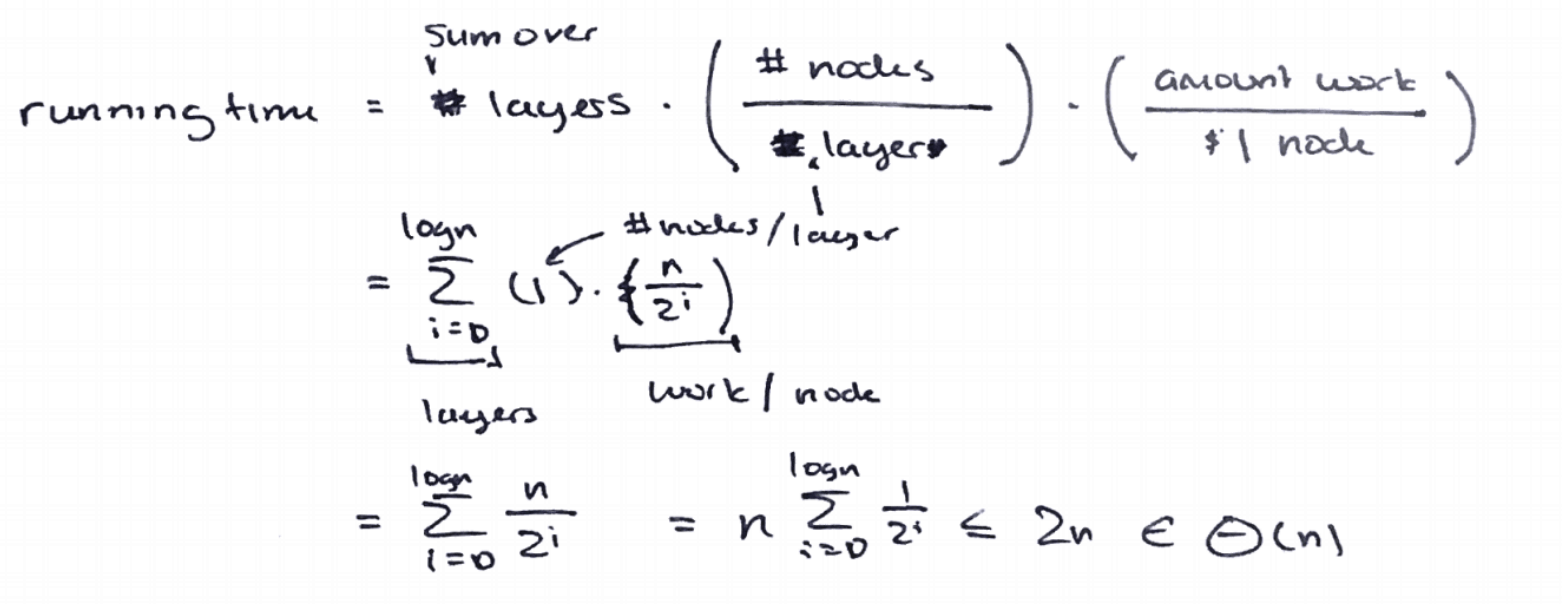### andwelcome(0, N)

# layer: $\log N$
# node: $1$ or $2^i$
amount of work: N (all nodes)

Worst case: $1 \times \frac{N}{1} + 2 \times \frac{N}{2} + 4 \times \frac{N}{4} … + N \times \frac{N}{N} \sim N \times \log(N) \in \Theta(N \log N)$
Best case: $N + \frac{N}{2} + \frac{N}{4} + … + 1 \sim \Theta(N)$

Note: If amount of work equals 1, running time will be $\Theta(\log N), just like the running time of binary search.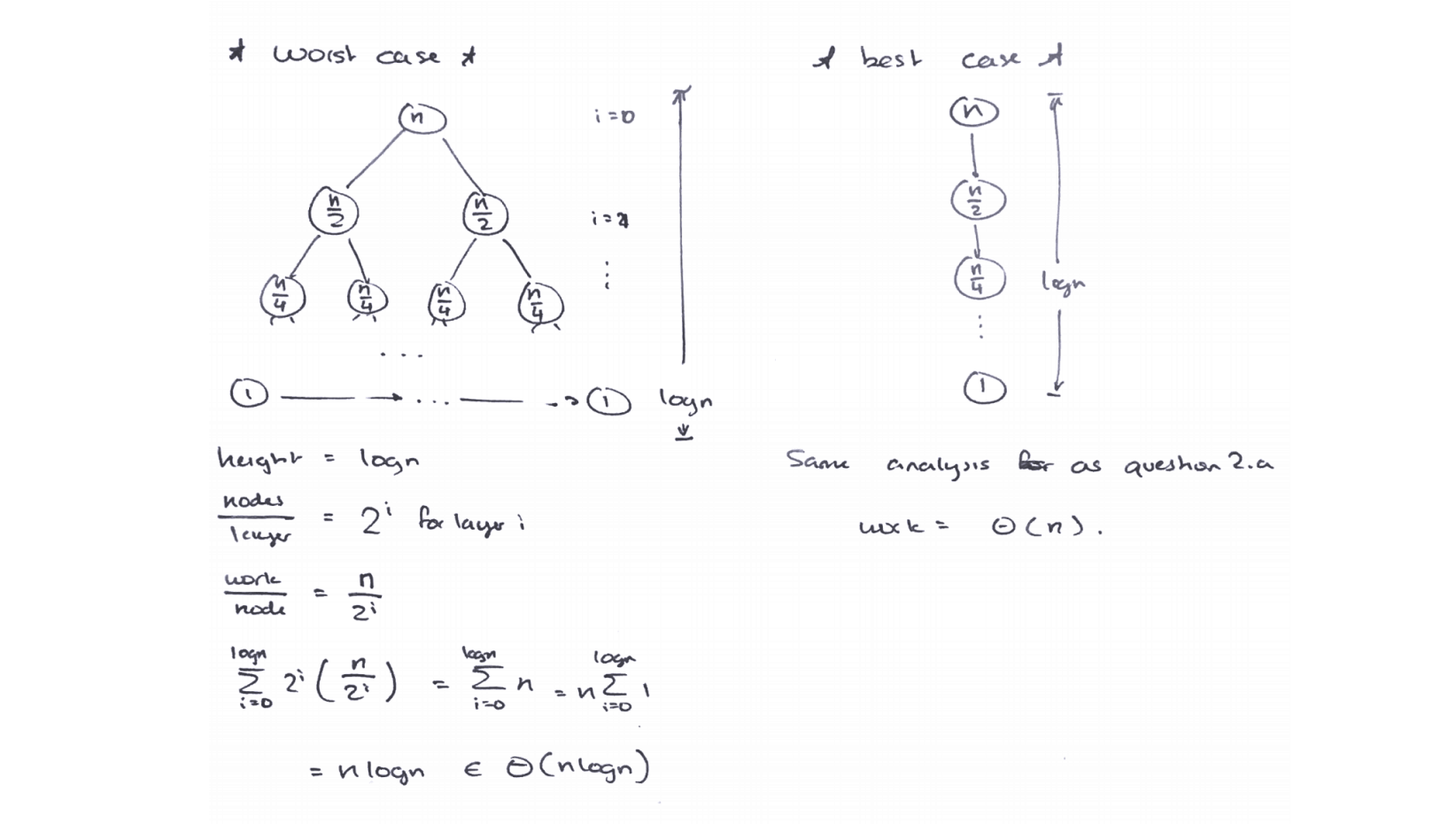### tothe Cost model: call to tothe() # layer:$N$# node:$2^i$amount of work:$O(1)$Worst case:$1 + 2 + 4 + 8 + … + 2^N \sim 2^N \times 2 - 1 \in \Theta(2^N)$Best case:$\Theta(2^N)$(same) ### spacejam (big O notation) # layer:$N$# node:$\frac{N!}{(N - i)!}$i = 0: 1 i = 1: N i = 2: (N - 1) x N i = 3: (N - 2) x (N - 1) x N i = 4: (N - 3) x (N - 2) x (N - 1) x N i = N: N! amount of work:$O(1)$Worst case:$\sum^{N}_0 \frac{N!}{(N - i)!}\times 1 \sim N \times N! \in O(N \times N!)$Best case:$O(N \times N!)$Actually, calculating the sum of$\frac{N!}{(N-i)!}$is a bit tricky because there is a pesky$(N-i)!$term in the denominator. We can upper bound the sum by just removing the denominator, but in the strictest sense we would now have a big-O bound instead of big-theta. ## Ex (Examprep 07) Given the following function definitions, what is the worst-case runtime for p(N)? Assume h is a boolean function requiring constant time.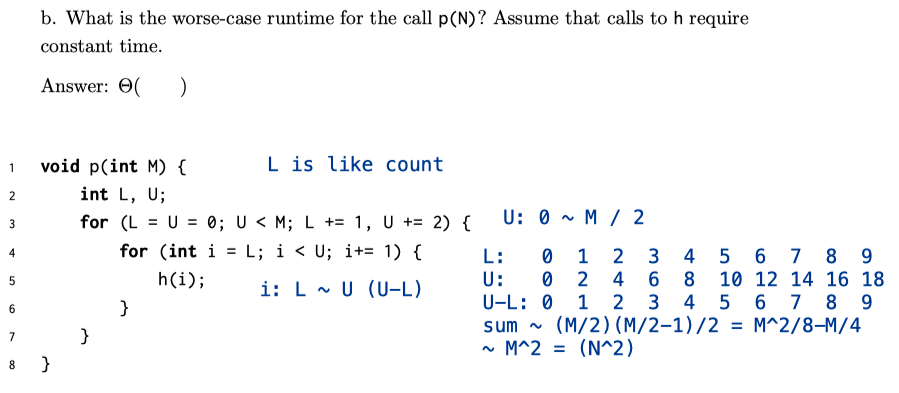## Disjoint Sets Two sets are named disjoint sets if they have no elements in common. A Disjoint-Sets (or Union-Find) data structure keeps track of a fixed number of elements partitioned into a number of disjoint sets. 1. connect(x, y): connect x and y. Also known as union 2. isConnected(x, y): returns true if x and y are connected (i.e. part of the same set). Goal: Design an efficient DisjointSets implementation. • Number of elements N can be huge. • Number of method calls M can be huge. • Calls to methods may be interspersed (invoking order). The Naive Approach (Approach Zero):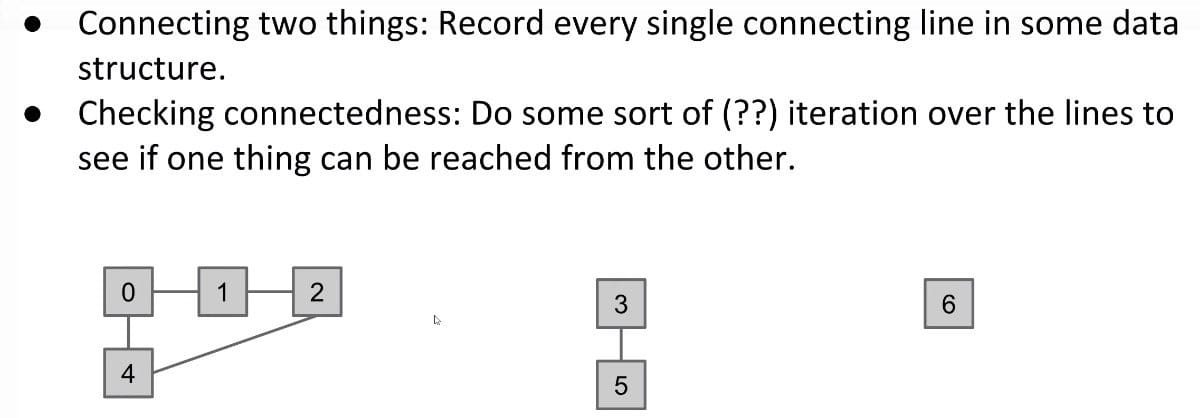The Better Approach: Connected Components. Rather than manually writing out every single connecting line, only record the sets that each item belongs to. In other words, we only need to keep track of which connected component each item belongs to. This chapter will be a chance to see how an implementation of a data structure evolves. We will discuss four iterations of a Disjoint Sets design before being satisfied: • ListOfSets • Quick Find • Quick Union • Weighted Quick Union (WQU) • WQU with Path Compression We will see how design decisions greatly affect asymptotic runtime and code complexity. ### ListOfSets Intuitively, we might first consider representing Disjoint Sets as a list of sets, e.g, List<Set<Integer>>. For instance, if we have N=6 elements and nothing has been connected yet, our list of sets looks like: [{0}, {1}, {2}, {3}, {4}, {5}, {6}]. Looks good. However, consider how to complete an operation like connect(5, 6). We’d have to iterate through up to N sets to find 5 and N sets to find 6. Our runtime becomes O(N). The lesson to take away is that initial design decisions determine our code complexity. ### Quick Find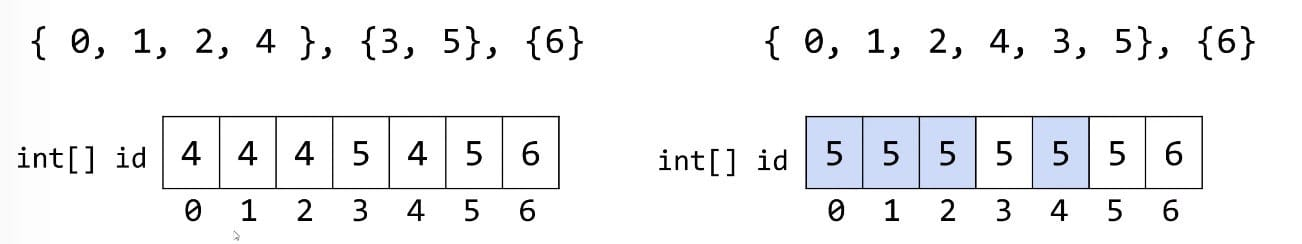Having to create N distinct sets initially is$\Theta(N)$.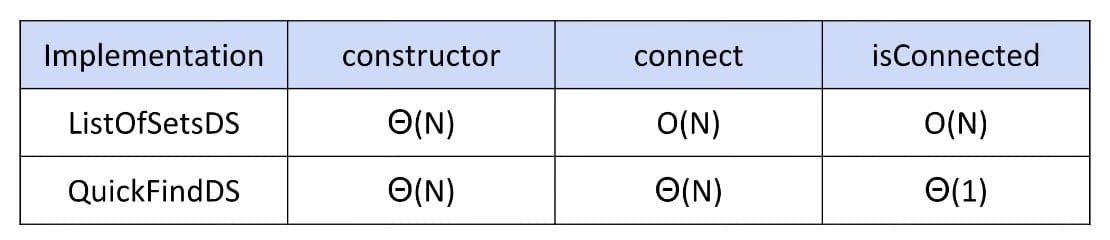### Quick Union Quick Find’s bad feature: Connecting two sets is slow! Difference: Values will be chosen so that connect is fast. Hard question: How could we change our set representation so that combining two sets into their union requires changing one value?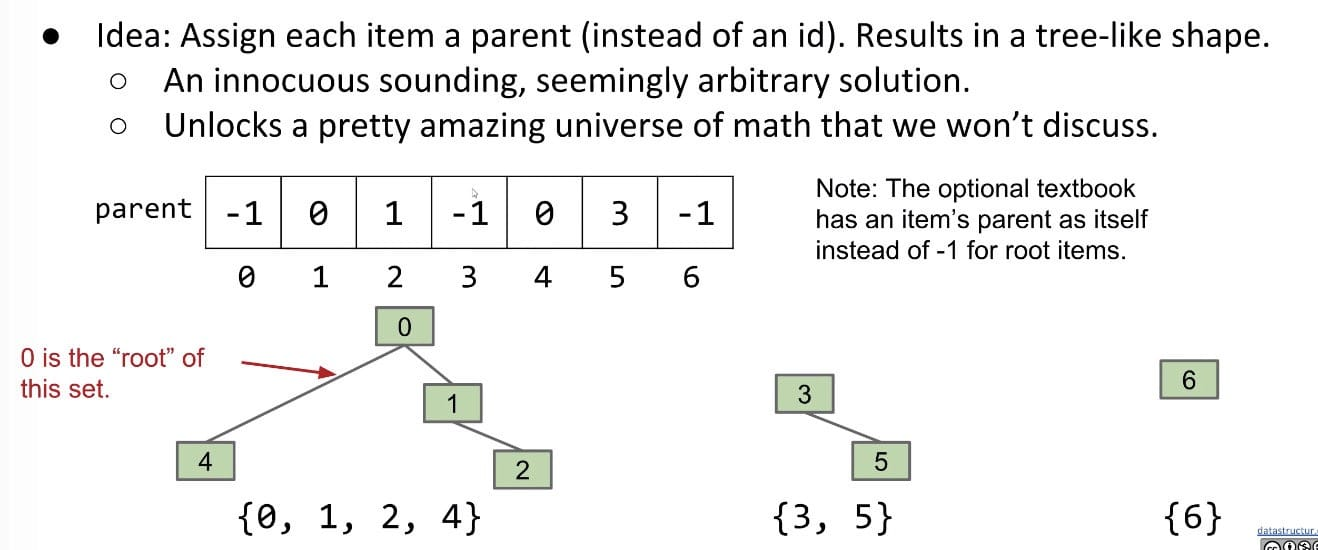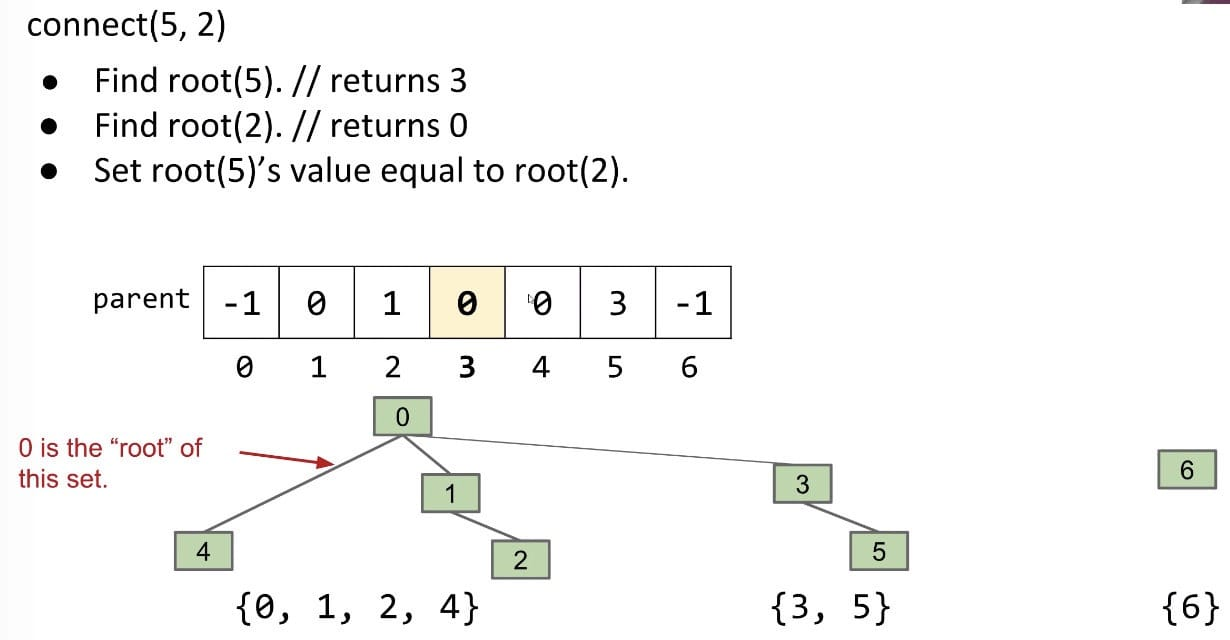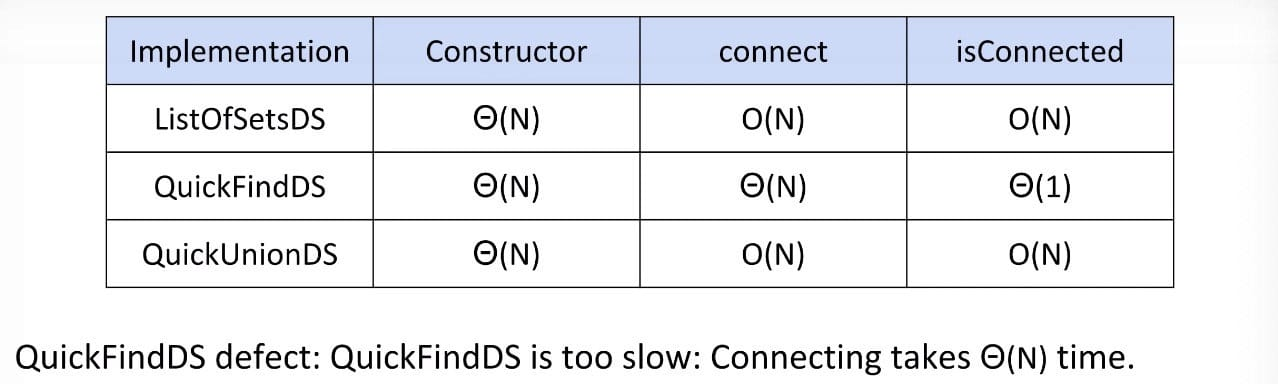QuickUnion defect: Trees can get tall. Results in potentially even worse performance than QuickFind if tree is imbalanced. Things would be find if we just kept our tree balanced. ### Weighted Quick Union (WQU)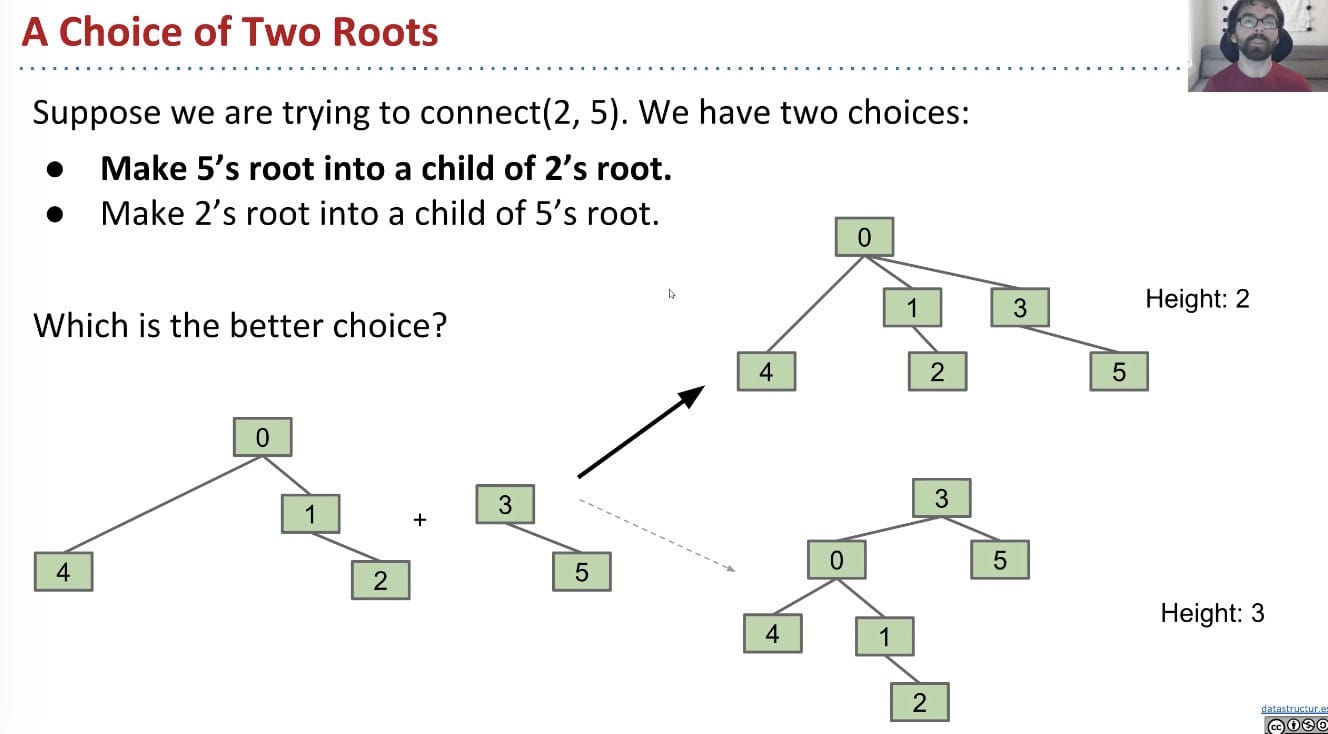Modify quick-union to avoid tall trees. • Track tree size (number of elements) • New Rule: Always link root of a smaller tree to a larger tree (weight, not height). connect(int p, int q) needs to somehow keep track of sizes. Two common approaches: 1. Use values other than -1 in parent array for root nodes to track size 2. Create a separate size array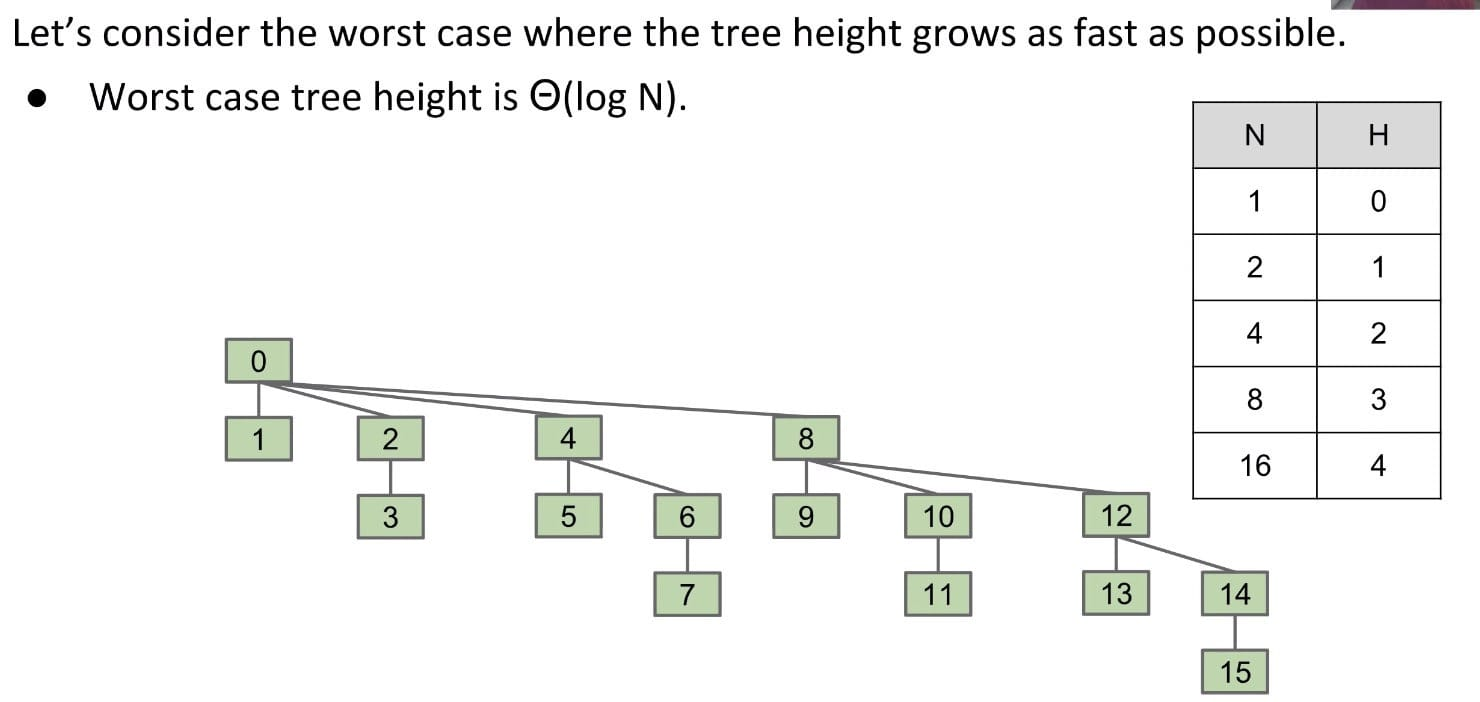Note: You can see if every time I just connect 1 item it would be great (the height keeps small).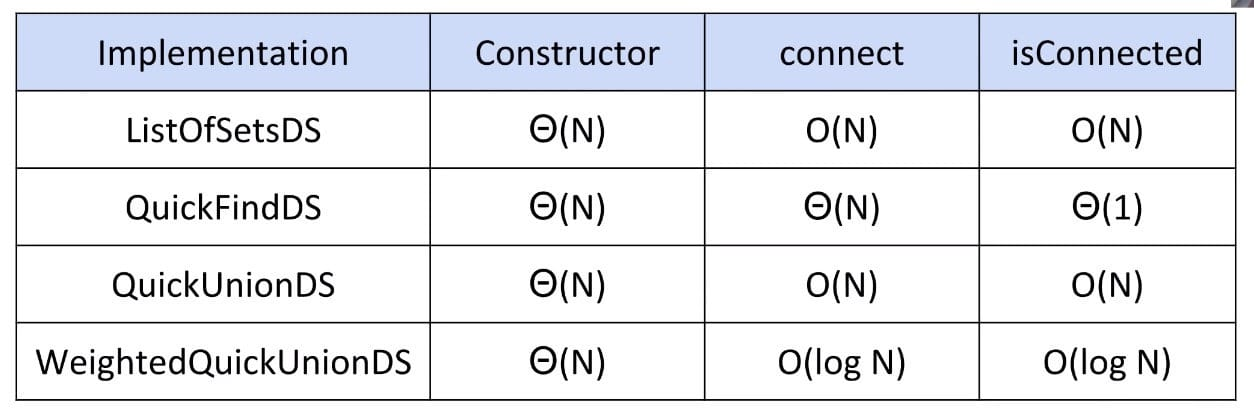### WQU with Path Compression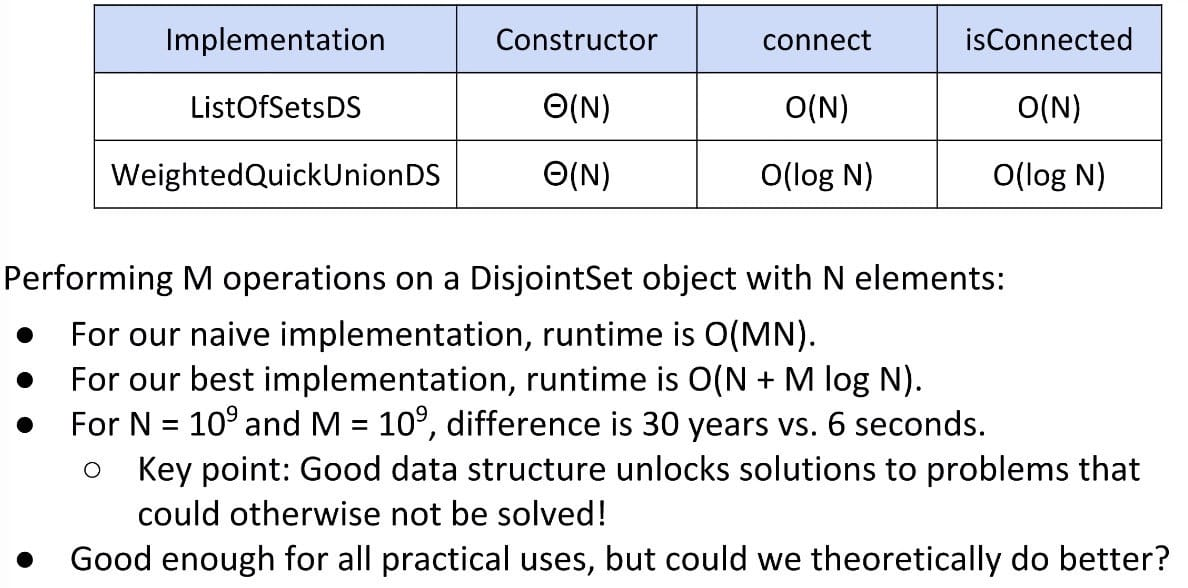Another clever idea when doing find: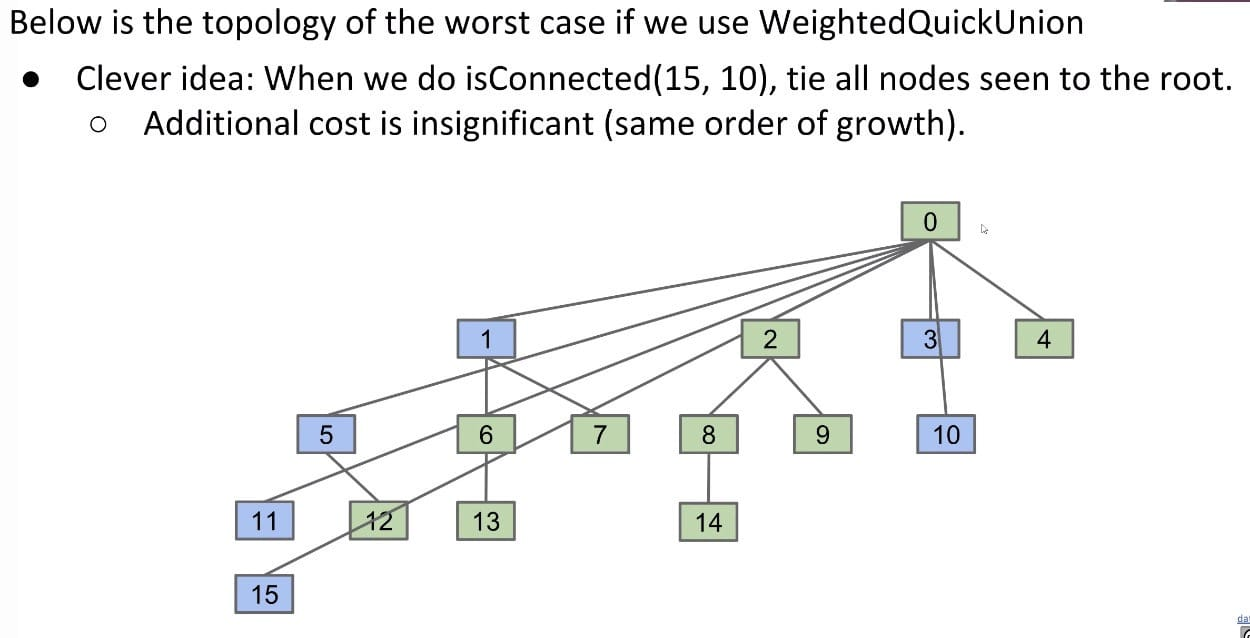Recall that both connect(x, y) and isConnected(x, y) always call find(x) and find(y). Thus, after calling connect or isConnected enough, essentially all elements will point directly to their root. By extension, the average runtime of connect and isConnected becomes almost constant in the long term! This is called the amortized runtime. Technically, the order of growth of this data structure becomes$O(N + M lg* N)$. lg* N, aka. the iterated logarithm, is less than 5 for any realistic input.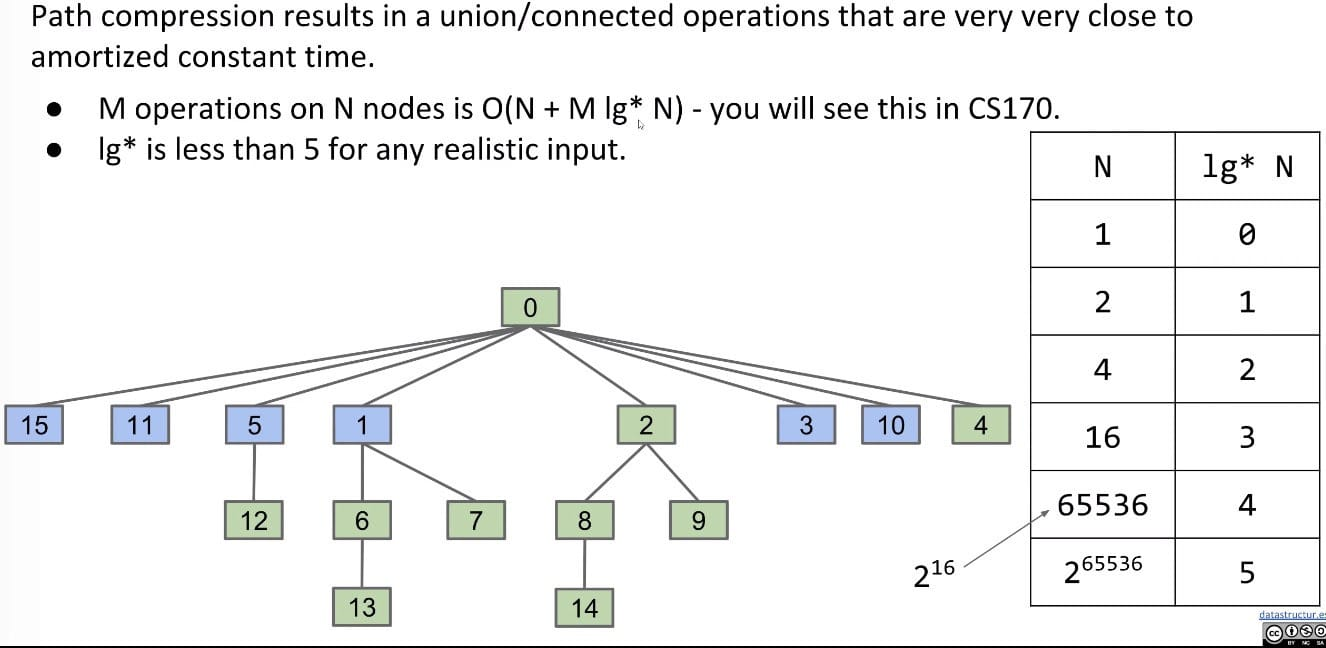For$lg* N$, to get up to 4,$N$needs to be$2^16$. Actually, there is a tighter bound:$O(N + M\alpha(N))$, where$\alpha$is the inverse Ackermann function which is less than 5 for all practical inputs! Done The end result of our iterative design process is the standard way disjoint sets are implemented today - quick union and path compression (once you have path compression, you don’t need weighted quick union).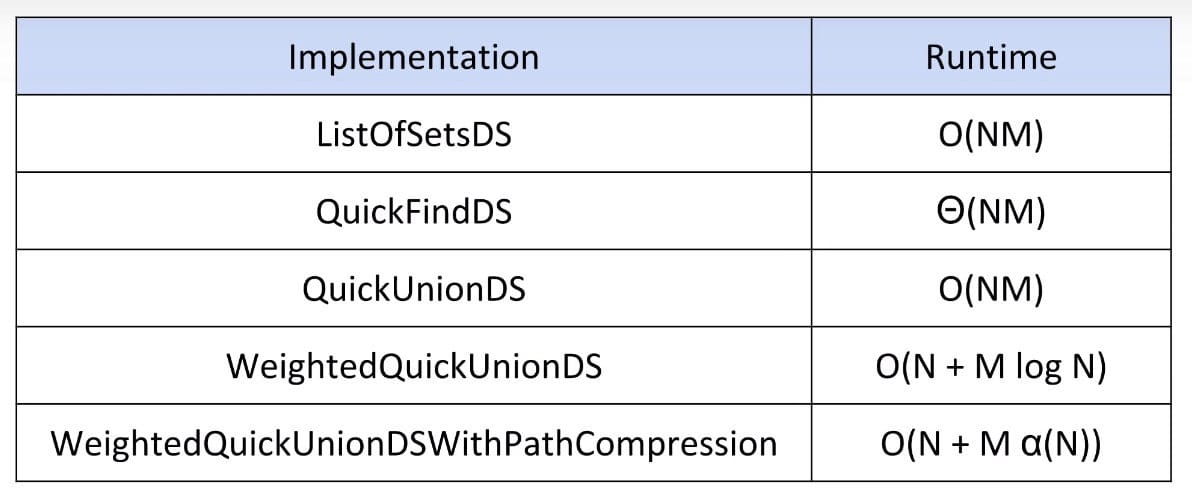### Implementation ### HW 2: Percolation HW 2: Percolation My Implementation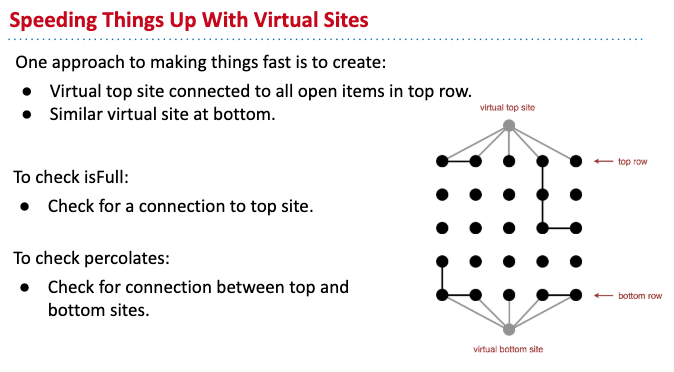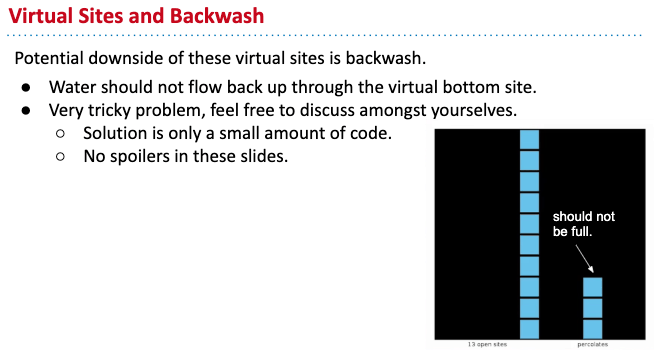Solution: Use 2 WeightedQuickUnionUFs (one with only top entry, the other one with both top and bottom entries) Possible Solution (Online): One additional N by N grid to store states ## Notes on Algorithms (Coursera) An algorithm must be seen to be believed. — Donald Knuth Log million, lg(1,000,000), is approximately 20.$1 + \frac{1}{2} + \frac{1}{3} + … + \frac{1}{N} = \ln N\$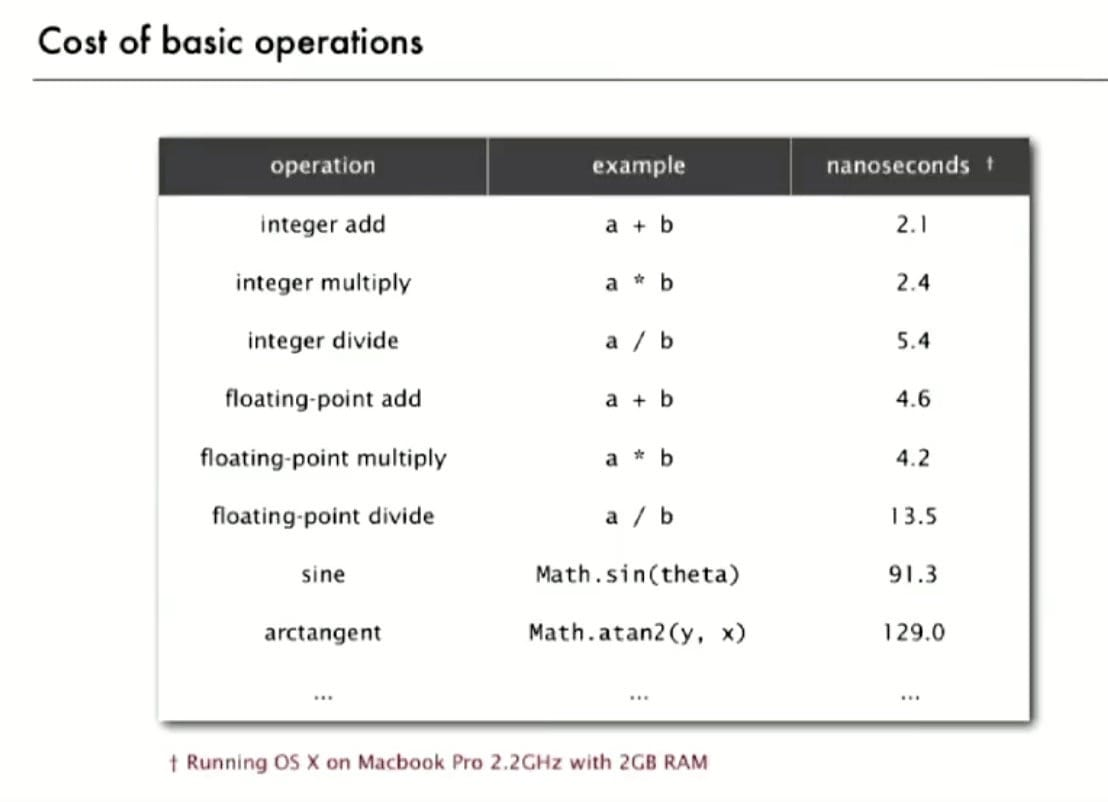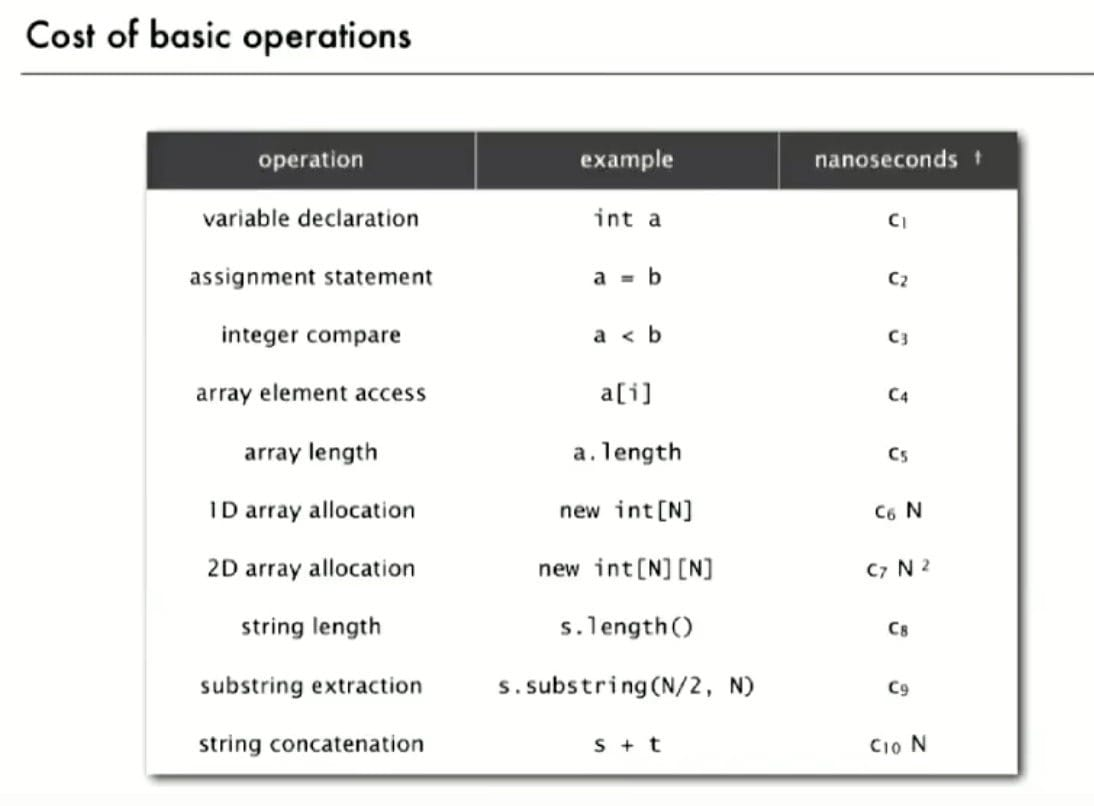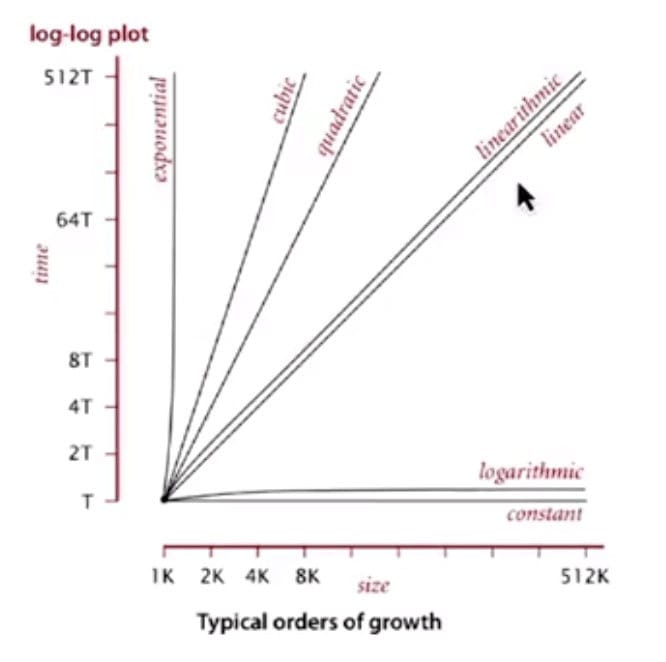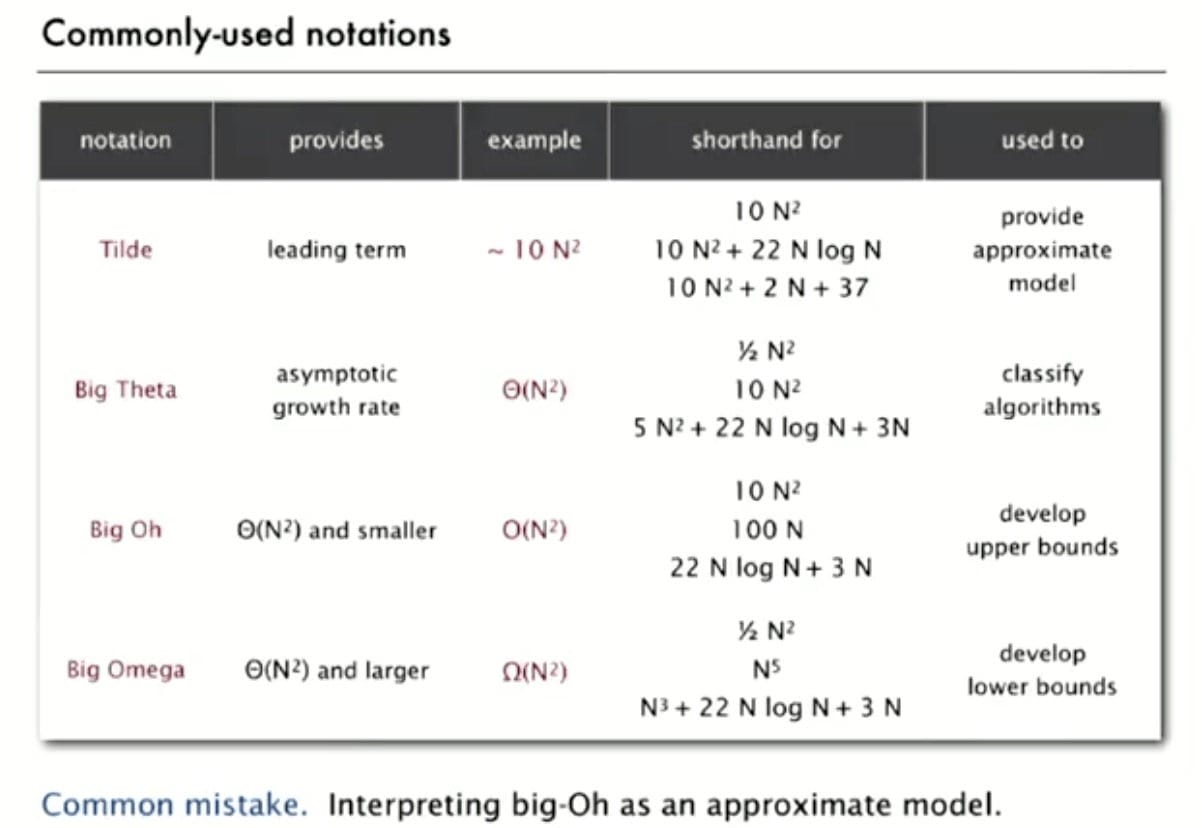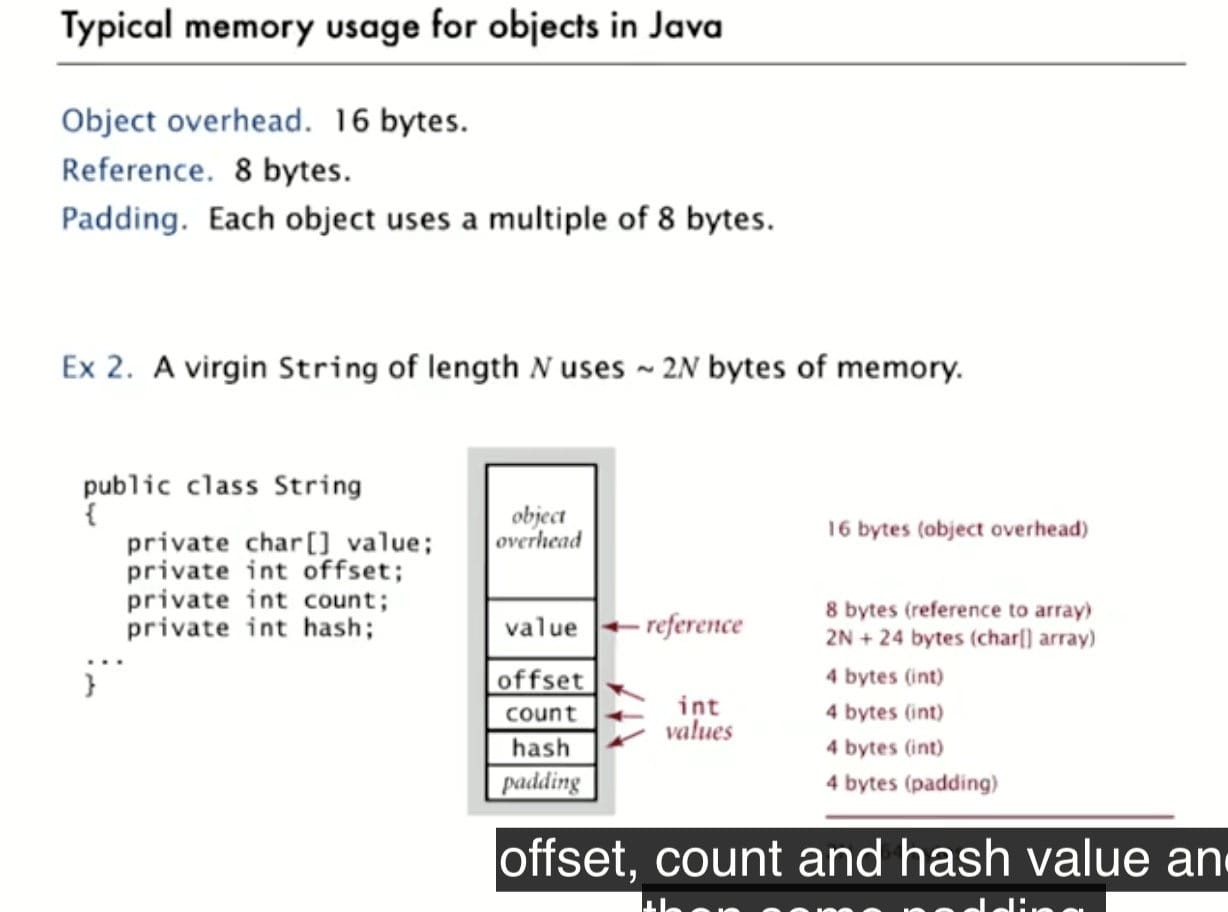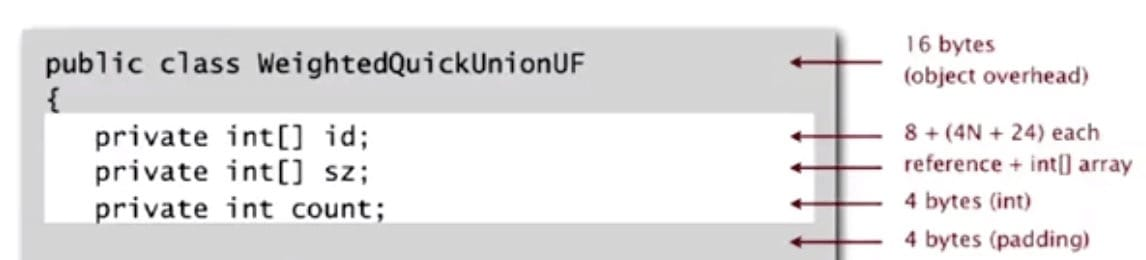0%